Скачать презентацию Machine Learning Machine a tour 1 -semester course

a39e5d40767c6f8d352664b82a1f72f7.ppt

• Количество слайдов: 81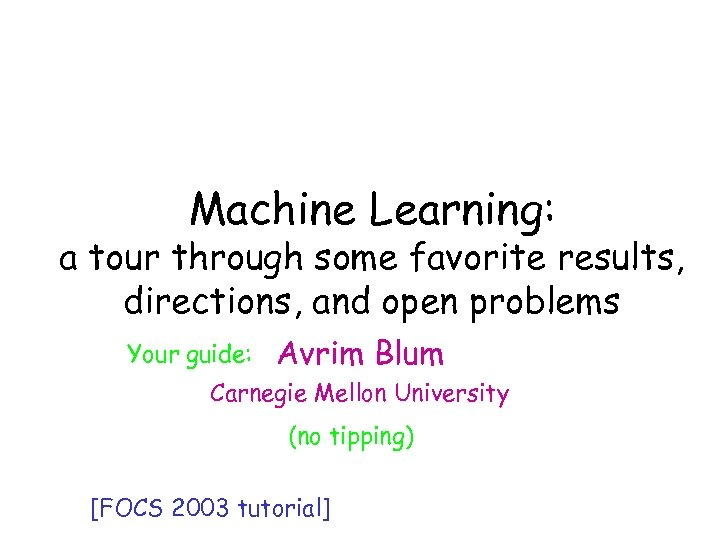Machine Learning: Machine a tour 1 -semester course in 2 hrs a through some favorite results, directions, and open problems Your guide: Avrim Blum Carnegie Mellon University (no tipping) [FOCS 2003 tutorial]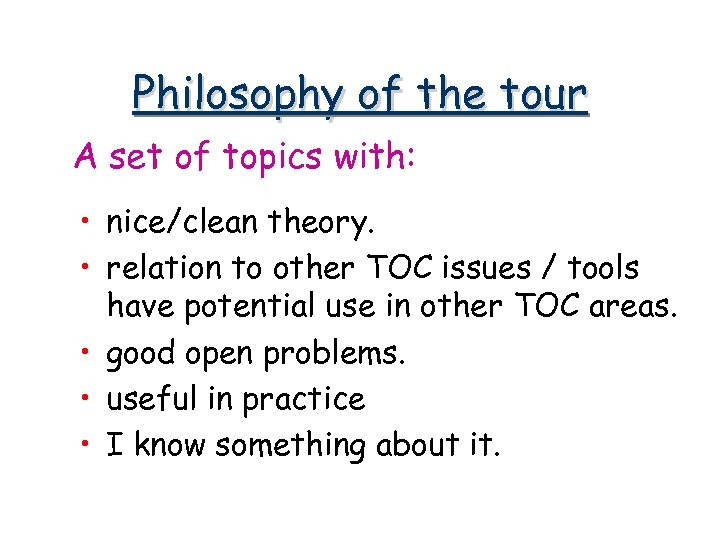Philosophy of the tour A set of topics with: • nice/clean theory. • relation to other TOC issues / tools have potential use in other TOC areas. • good open problems. • useful in practice • I know something about it.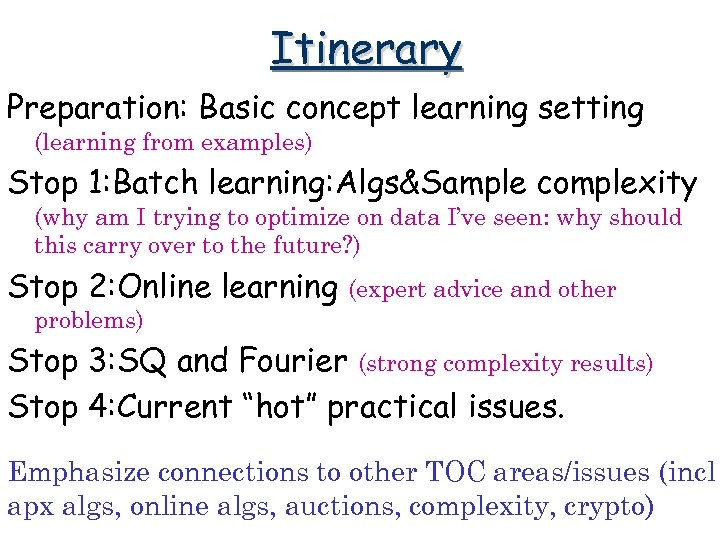Itinerary Preparation: Basic concept learning setting (learning from examples) Stop 1: Batch learning: Algs&Sample complexity (why am I trying to optimize on data I’ve seen: why should this carry over to the future? ) Stop 2: Online learning (expert advice and other problems) Stop 3: SQ and Fourier (strong complexity results) Stop 4: Current “hot” practical issues. Emphasize connections to other TOC areas/issues (incl apx algs, online algs, auctions, complexity, crypto)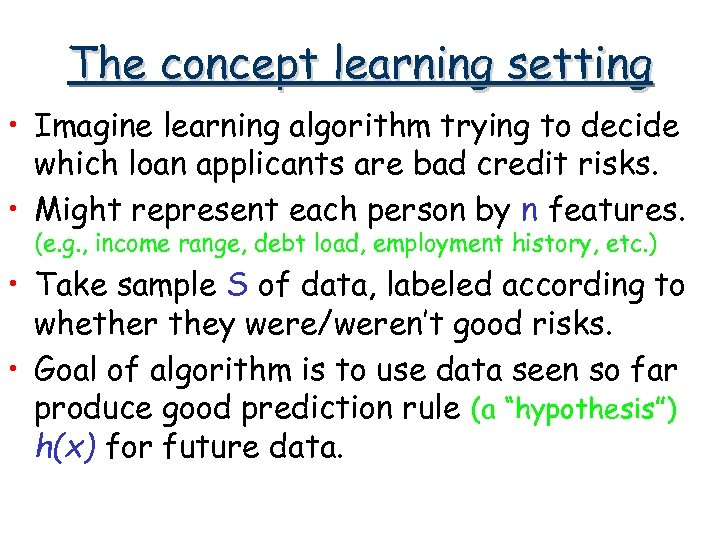The concept learning setting • Imagine learning algorithm trying to decide which loan applicants are bad credit risks. • Might represent each person by n features. (e. g. , income range, debt load, employment history, etc. ) • Take sample S of data, labeled according to whether they were/weren’t good risks. • Goal of algorithm is to use data seen so far produce good prediction rule (a “hypothesis”) h(x) for future data.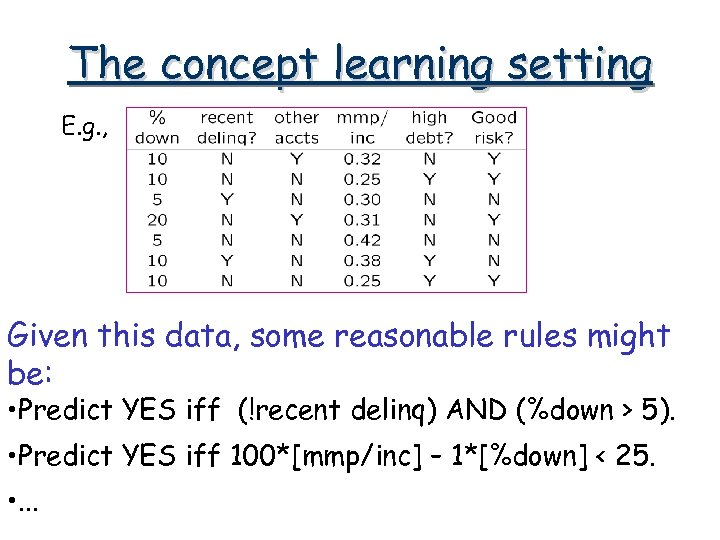The concept learning setting E. g. , Given this data, some reasonable rules might be: • Predict YES iff (!recent delinq) AND (%down > 5). • Predict YES iff 100*[mmp/inc] – 1*[%down] < 25. • . . .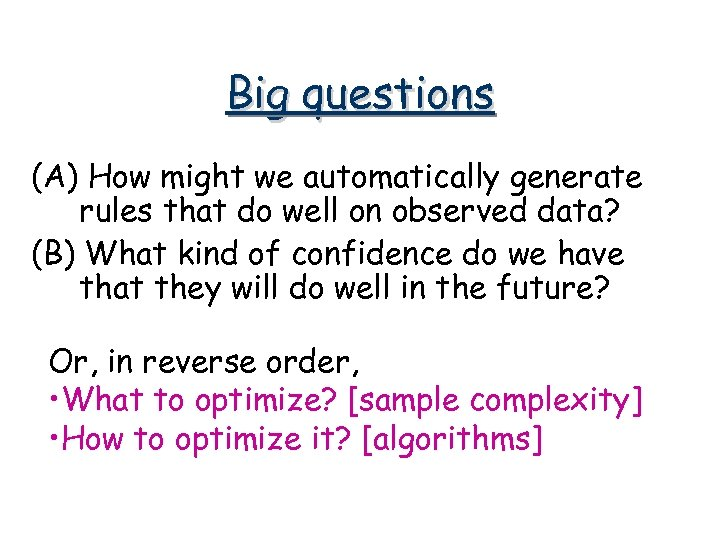Big questions (A) How might we automatically generate rules that do well on observed data? (B) What kind of confidence do we have that they will do well in the future? Or, in reverse order, • What to optimize? [sample complexity] • How to optimize it? [algorithms]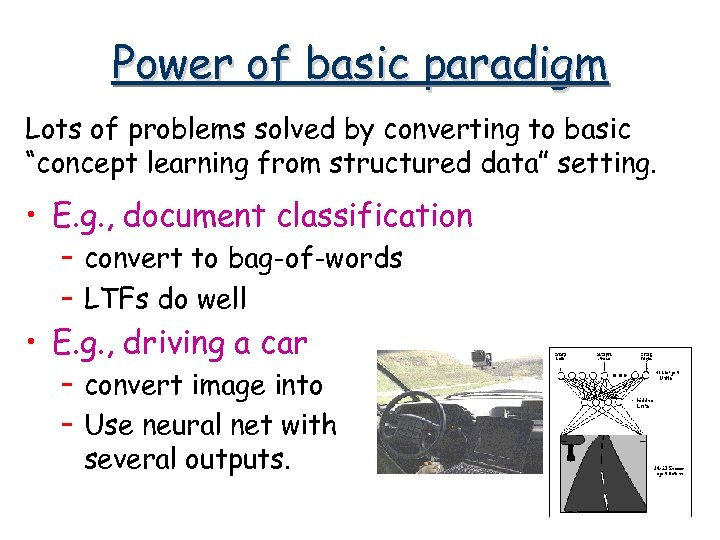Power of basic paradigm Lots of problems solved by converting to basic “concept learning from structured data” setting. • E. g. , document classification – convert to bag-of-words – LTFs do well • E. g. , driving a car – convert image into features. – Use neural net with several outputs.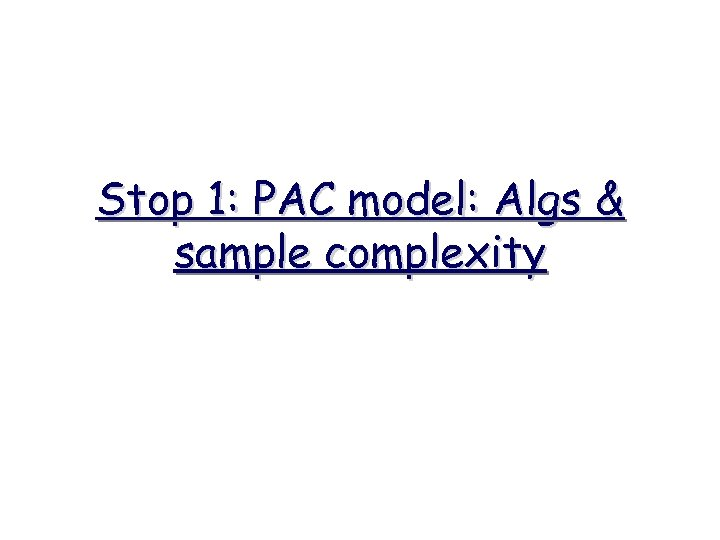Stop 1: PAC model: Algs & sample complexity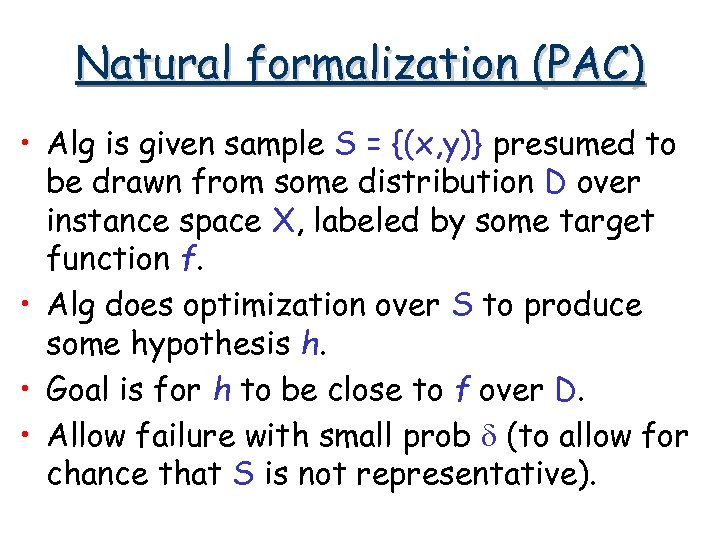Natural formalization (PAC) • Alg is given sample S = {(x, y)} presumed to be drawn from some distribution D over instance space X, labeled by some target function f. • Alg does optimization over S to produce some hypothesis h. • Goal is for h to be close to f over D. • Allow failure with small prob d (to allow for chance that S is not representative).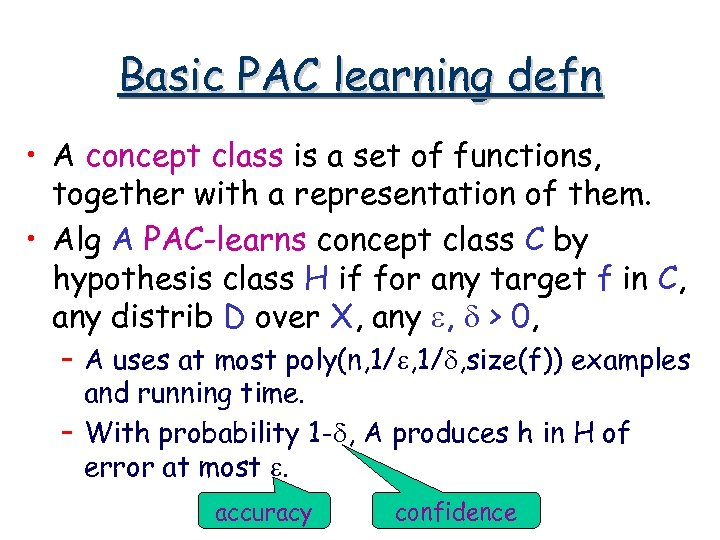Basic PAC learning defn • A concept class is a set of functions, together with a representation of them. • Alg A PAC-learns concept class C by hypothesis class H if for any target f in C, any distrib D over X, any e, d > 0, – A uses at most poly(n, 1/e, 1/d, size(f)) examples and running time. – With probability 1 -d, A produces h in H of error at most e. accuracy confidence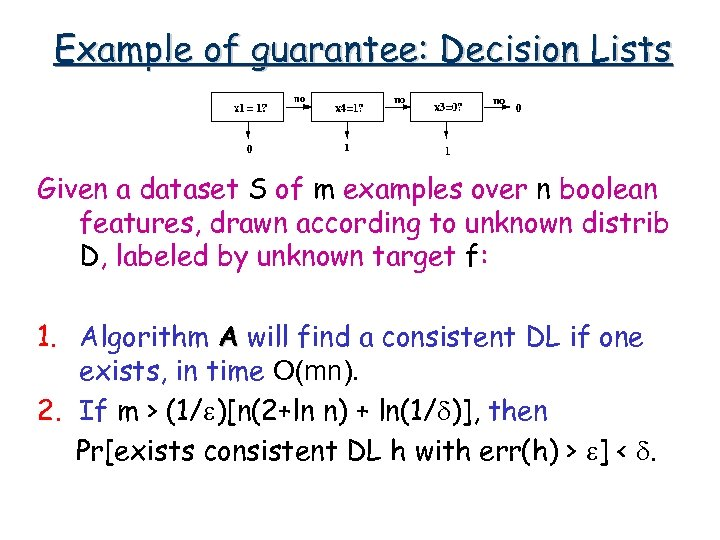Example of guarantee: Decision Lists Given a dataset S of m examples over n boolean features, drawn according to unknown distrib D, labeled by unknown target f: 1. Algorithm A will find a consistent DL if one exists, in time O(mn). 2. If m > (1/e)[n(2+ln n) + ln(1/d)], then Pr[exists consistent DL h with err(h) > e] < d.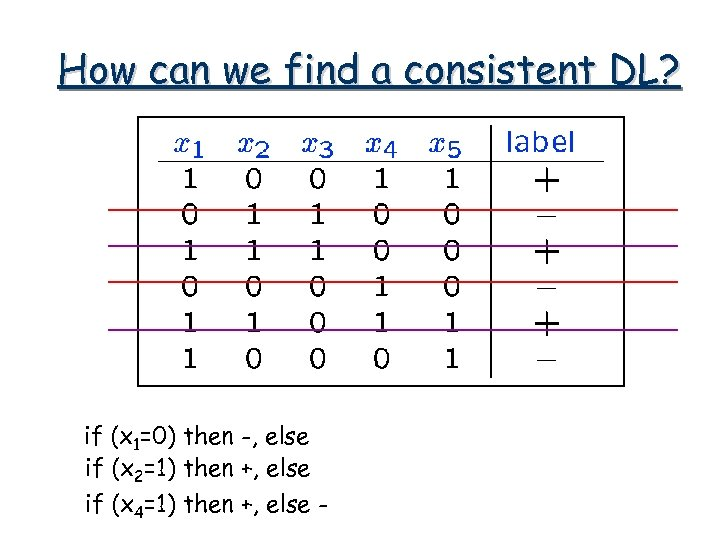How can we find a consistent DL? if (x 1=0) then -, else if (x 2=1) then +, else if (x 4=1) then +, else -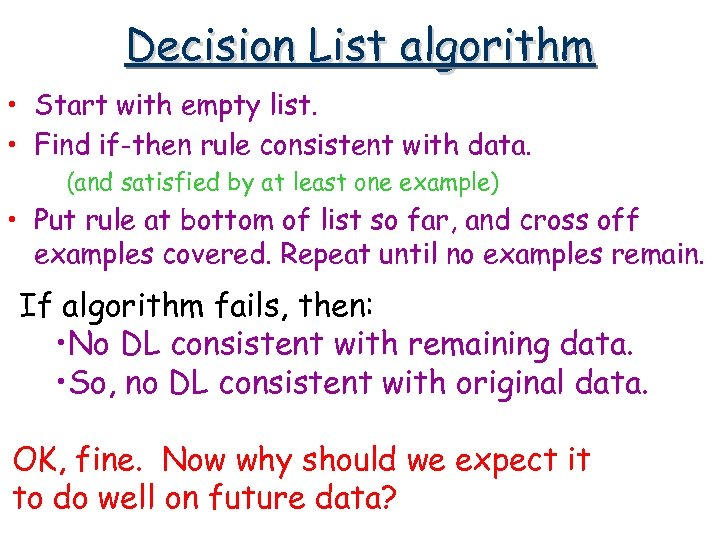Decision List algorithm • Start with empty list. • Find if-then rule consistent with data. (and satisfied by at least one example) • Put rule at bottom of list so far, and cross off examples covered. Repeat until no examples remain. If algorithm fails, then: • No DL consistent with remaining data. • So, no DL consistent with original data. OK, fine. Now why should we expect it to do well on future data?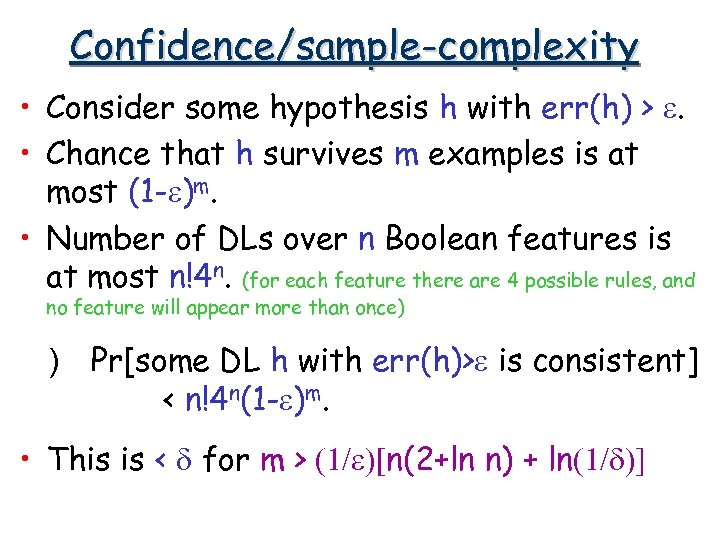Confidence/sample-complexity • Consider some hypothesis h with err(h) > e. • Chance that h survives m examples is at most (1 -e)m. • Number of DLs over n Boolean features is at most n!4 n. (for each feature there are 4 possible rules, and no feature will appear more than once) ) Pr[some DL h with err(h)>e is consistent] < n!4 n(1 -e)m. • This is < d for m > (1/e)[n(2+ln n) + ln(1/d)]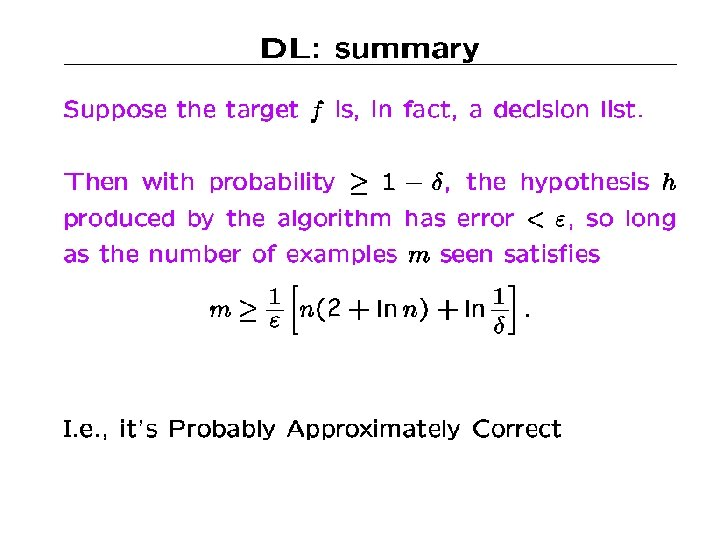2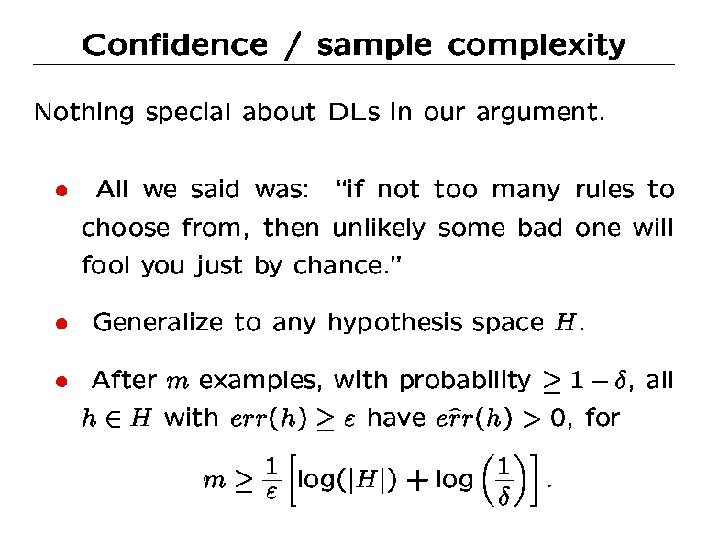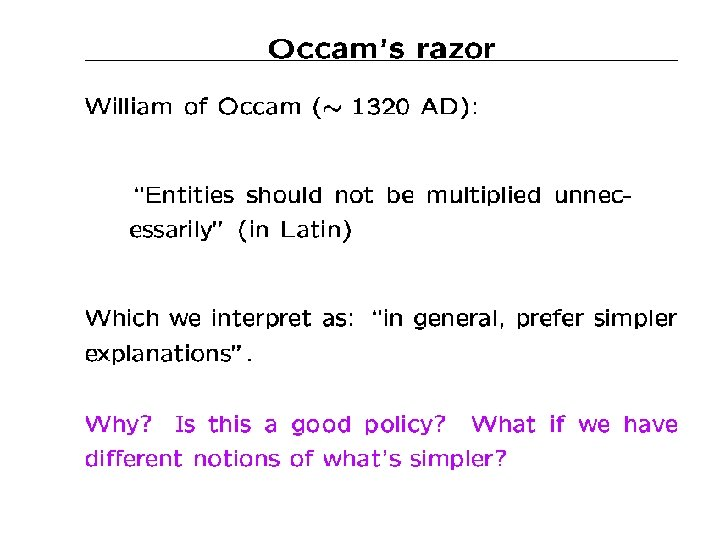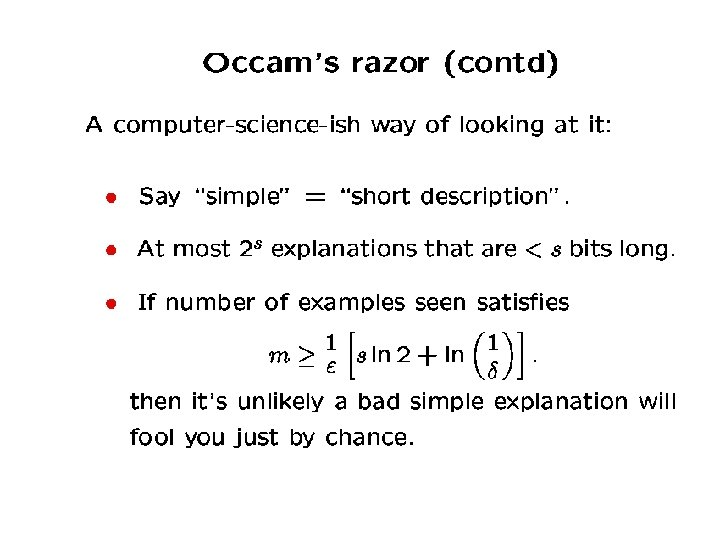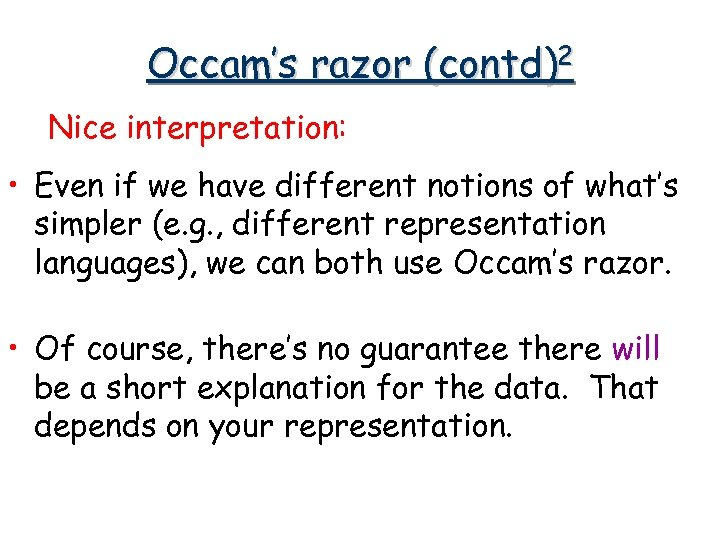Occam’s razor (contd)2 Nice interpretation: • Even if we have different notions of what’s simpler (e. g. , different representation languages), we can both use Occam’s razor. • Of course, there’s no guarantee there will be a short explanation for the data. That depends on your representation.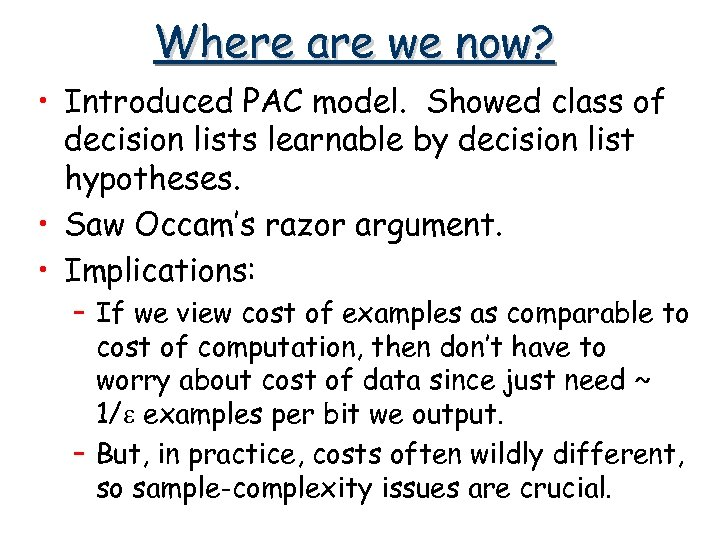Where are we now? • Introduced PAC model. Showed class of decision lists learnable by decision list hypotheses. • Saw Occam’s razor argument. • Implications: – If we view cost of examples as comparable to cost of computation, then don’t have to worry about cost of data since just need ~ 1/e examples per bit we output. – But, in practice, costs often wildly different, so sample-complexity issues are crucial.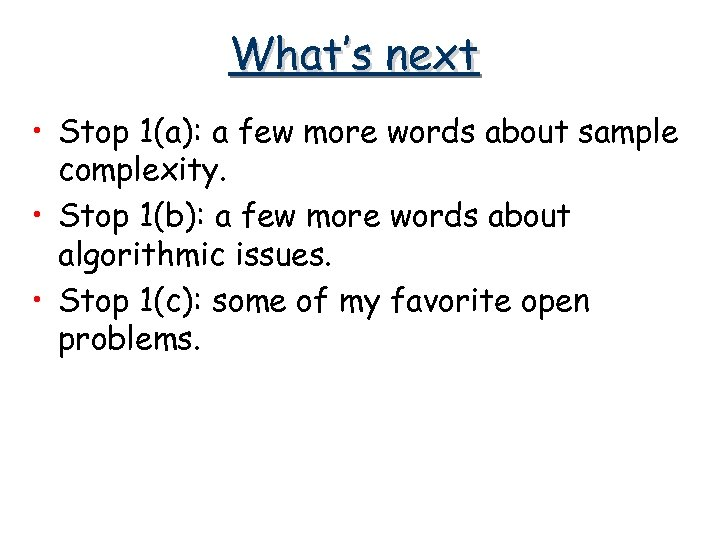What’s next • Stop 1(a): a few more words about sample complexity. • Stop 1(b): a few more words about algorithmic issues. • Stop 1(c): some of my favorite open problems.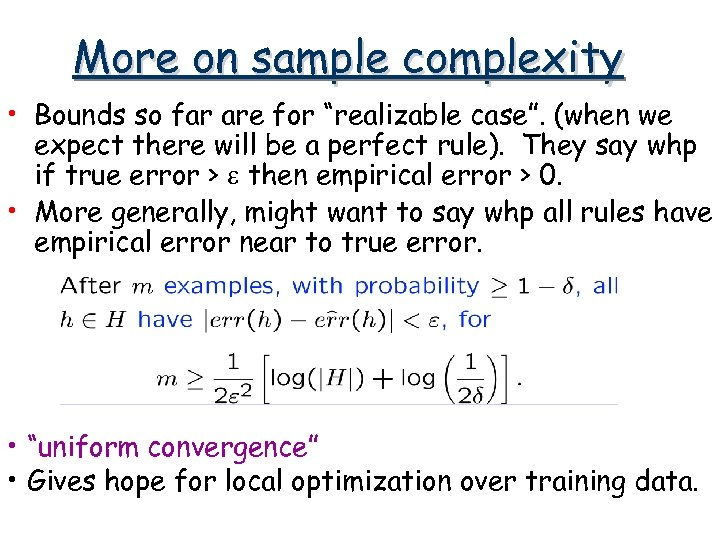More on sample complexity • Bounds so far are for “realizable case”. (when we expect there will be a perfect rule). They say whp if true error > e then empirical error > 0. • More generally, might want to say whp all rules have empirical error near to true error. • “uniform convergence” • Gives hope for local optimization over training data.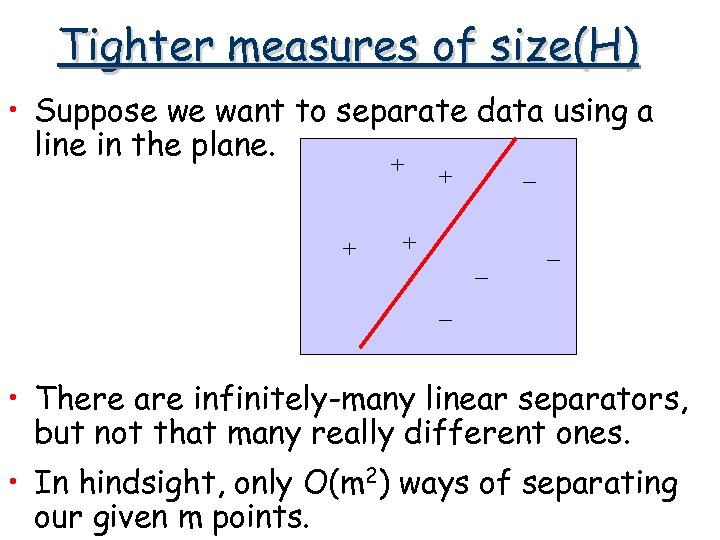Tighter measures of size(H) • Suppose we want to separate data using a line in the plane. + + + - - - • There are infinitely-many linear separators, but not that many really different ones. • In hindsight, only O(m 2) ways of separating our given m points.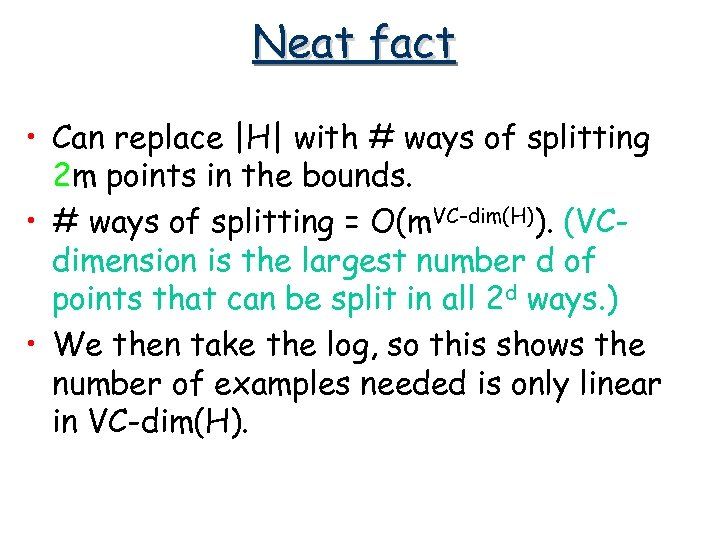Neat fact • Can replace |H| with # ways of splitting 2 m points in the bounds. • # ways of splitting = O(m. VC-dim(H)). (VCdimension is the largest number d of points that can be split in all 2 d ways. ) • We then take the log, so this shows the number of examples needed is only linear in VC-dim(H).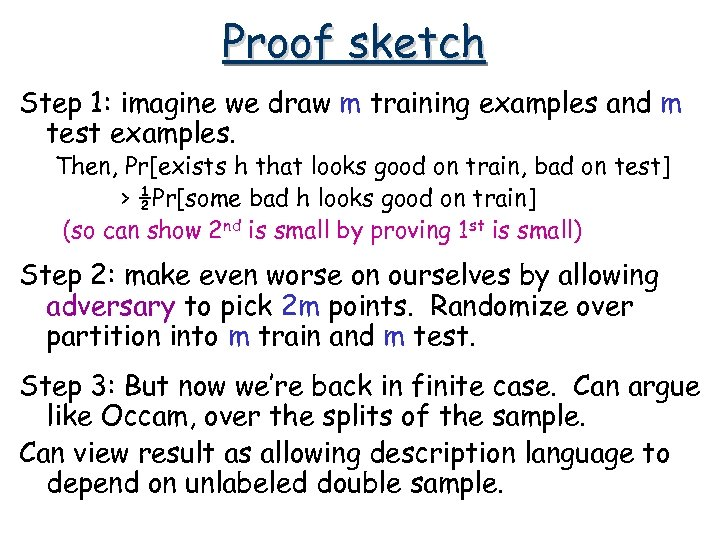Proof sketch Step 1: imagine we draw m training examples and m test examples. Then, Pr[exists h that looks good on train, bad on test] > ½Pr[some bad h looks good on train] (so can show 2 nd is small by proving 1 st is small) Step 2: make even worse on ourselves by allowing adversary to pick 2 m points. Randomize over partition into m train and m test. Step 3: But now we’re back in finite case. Can argue like Occam, over the splits of the sample. Can view result as allowing description language to depend on unlabeled double sample.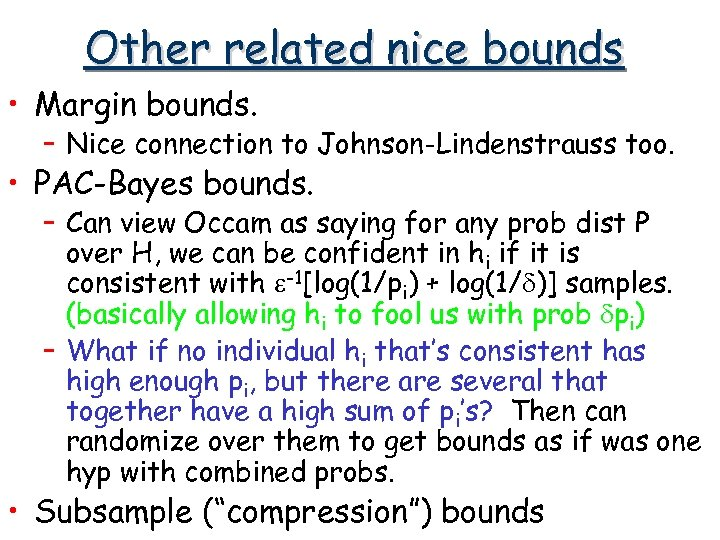Other related nice bounds • Margin bounds. – Nice connection to Johnson-Lindenstrauss too. • PAC-Bayes bounds. – Can view Occam as saying for any prob dist P over H, we can be confident in hi if it is consistent with e-1[log(1/pi) + log(1/d)] samples. (basically allowing hi to fool us with prob dpi) – What if no individual hi that’s consistent has high enough pi, but there are several that together have a high sum of pi’s? Then can randomize over them to get bounds as if was one hyp with combined probs. • Subsample (“compression”) bounds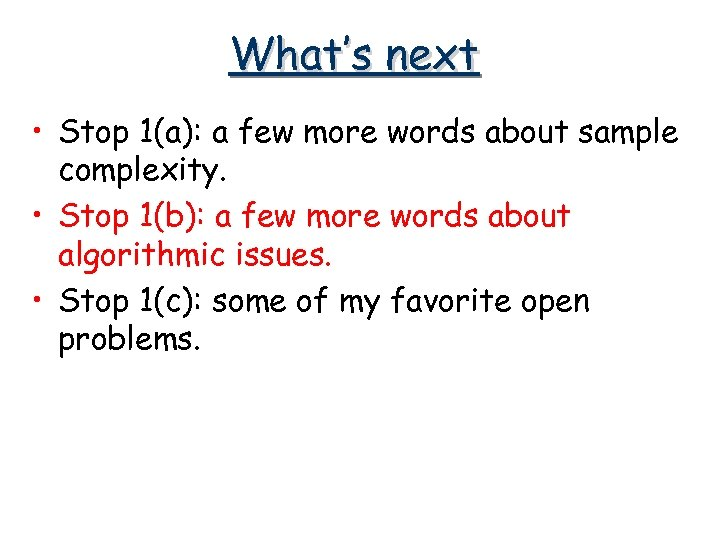What’s next • Stop 1(a): a few more words about sample complexity. • Stop 1(b): a few more words about algorithmic issues. • Stop 1(c): some of my favorite open problems.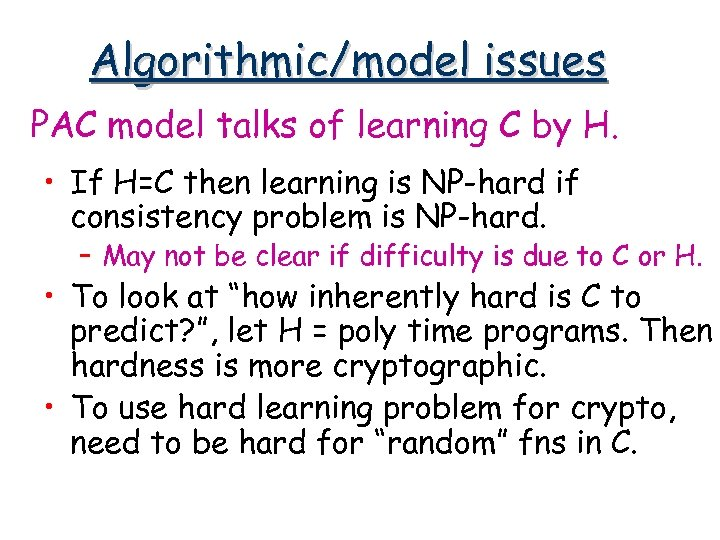Algorithmic/model issues PAC model talks of learning C by H. • If H=C then learning is NP-hard if consistency problem is NP-hard. – May not be clear if difficulty is due to C or H. • To look at “how inherently hard is C to predict? ”, let H = poly time programs. Then hardness is more cryptographic. • To use hard learning problem for crypto, need to be hard for “random” fns in C.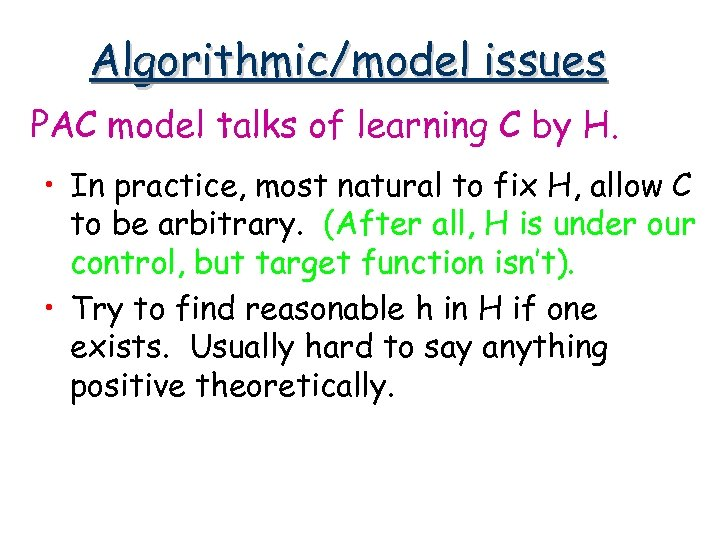Algorithmic/model issues PAC model talks of learning C by H. • In practice, most natural to fix H, allow C to be arbitrary. (After all, H is under our control, but target function isn’t). • Try to find reasonable h in H if one exists. Usually hard to say anything positive theoretically.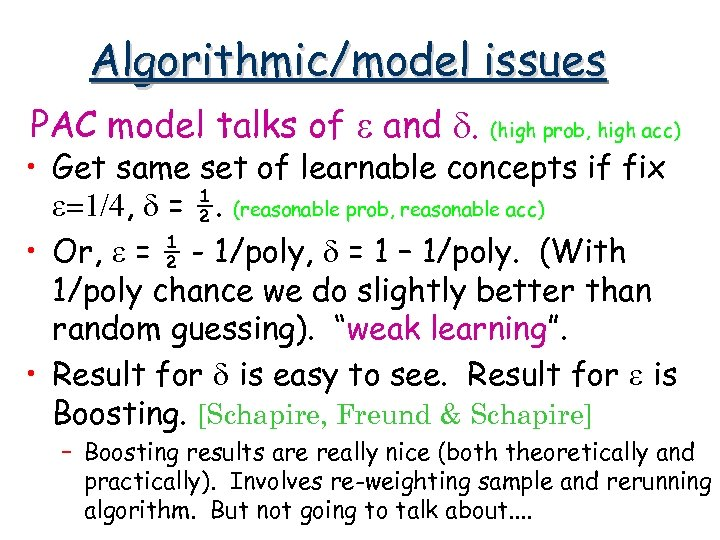Algorithmic/model issues PAC model talks of e and d. (high prob, high acc) • Get same set of learnable concepts if fix e=1/4, d = ½. (reasonable prob, reasonable acc) • Or, e = ½ - 1/poly, d = 1 – 1/poly. (With 1/poly chance we do slightly better than random guessing). “weak learning”. • Result for d is easy to see. Result for e is Boosting. [Schapire, Freund & Schapire] – Boosting results are really nice (both theoretically and practically). Involves re-weighting sample and rerunning algorithm. But not going to talk about. .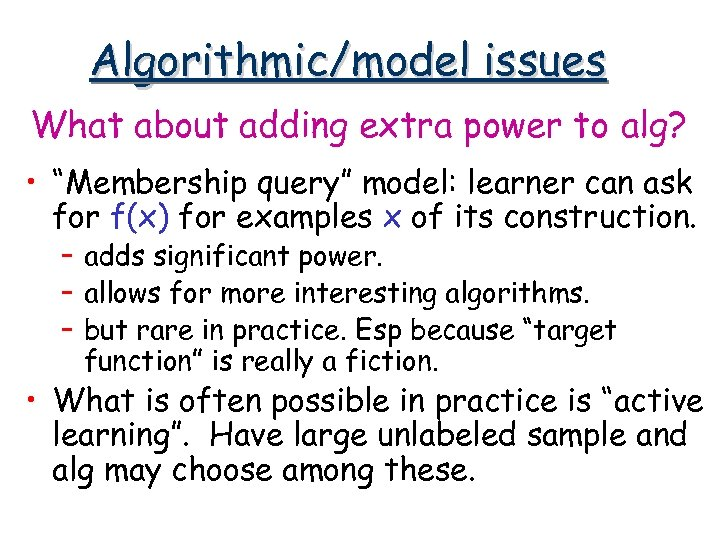Algorithmic/model issues What about adding extra power to alg? • “Membership query” model: learner can ask for f(x) for examples x of its construction. – adds significant power. – allows for more interesting algorithms. – but rare in practice. Esp because “target function” is really a fiction. • What is often possible in practice is “active learning”. Have large unlabeled sample and alg may choose among these.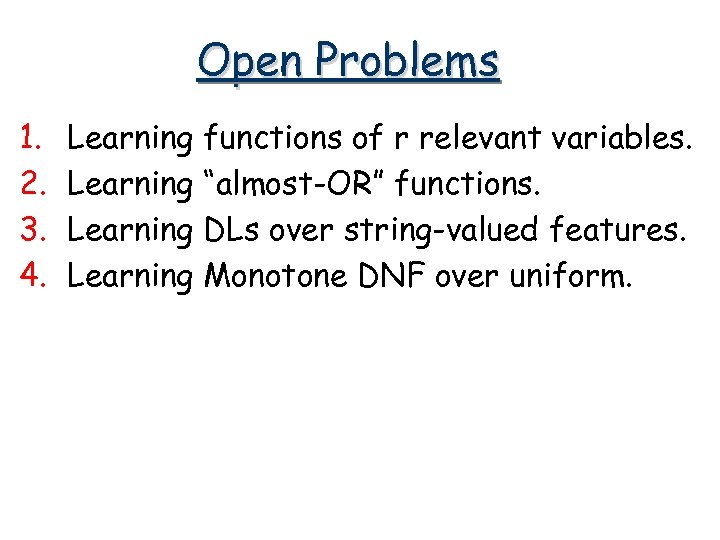Open Problems 1. 2. 3. 4. Learning functions of r relevant variables. Learning “almost-OR” functions. Learning DLs over string-valued features. Learning Monotone DNF over uniform.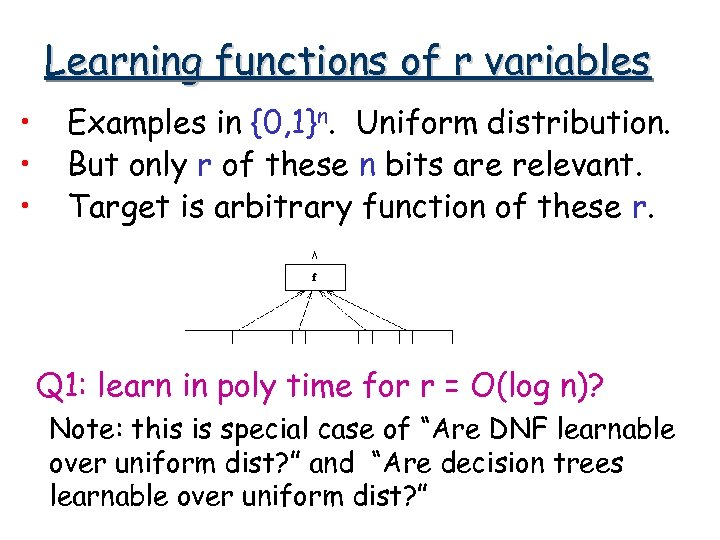Learning functions of r variables • • • Examples in {0, 1}n. Uniform distribution. But only r of these n bits are relevant. Target is arbitrary function of these r. Q 1: learn in poly time for r = O(log n)? Note: this is special case of “Are DNF learnable over uniform dist? ” and “Are decision trees learnable over uniform dist? ”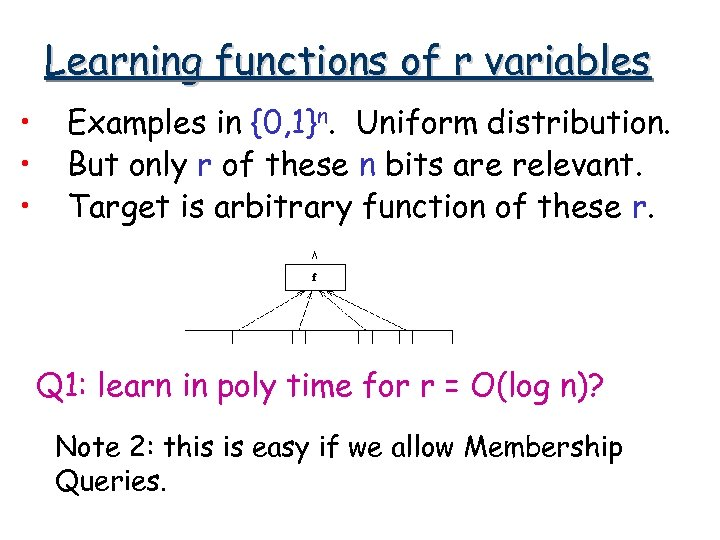Learning functions of r variables • • • Examples in {0, 1}n. Uniform distribution. But only r of these n bits are relevant. Target is arbitrary function of these r. Q 1: learn in poly time for r = O(log n)? Note 2: this is easy if we allow Membership Queries.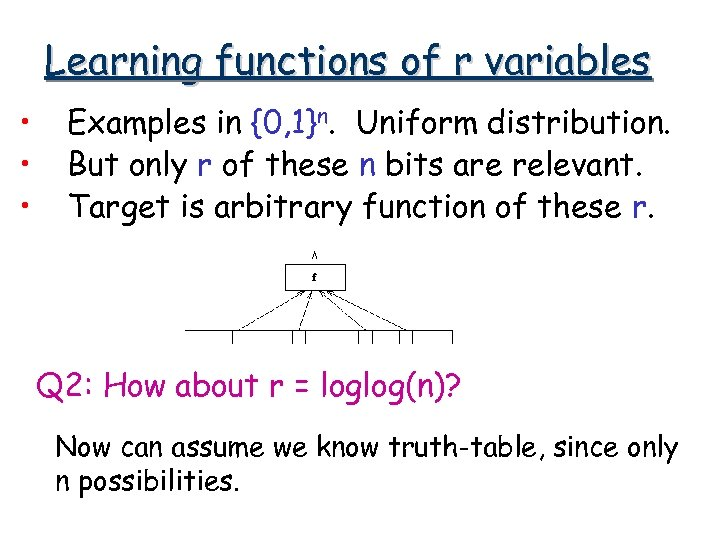Learning functions of r variables • • • Examples in {0, 1}n. Uniform distribution. But only r of these n bits are relevant. Target is arbitrary function of these r. Q 2: How about r = loglog(n)? Now can assume we know truth-table, since only n possibilities.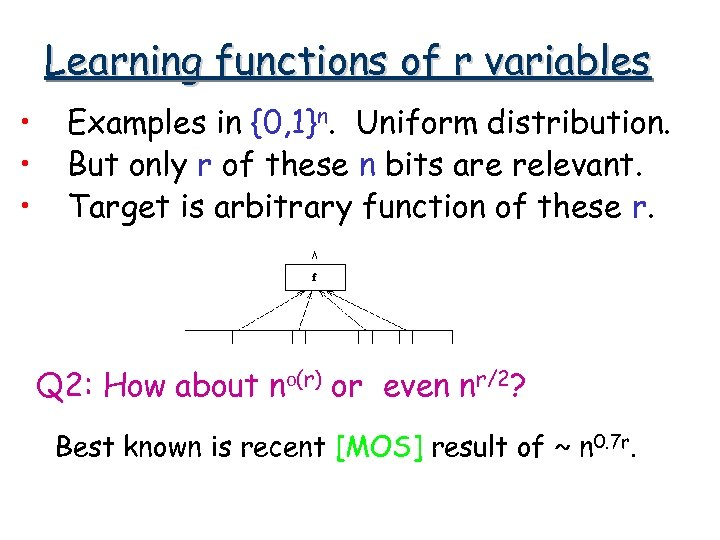Learning functions of r variables • • • Examples in {0, 1}n. Uniform distribution. But only r of these n bits are relevant. Target is arbitrary function of these r. Q 2: How about no(r) or even nr/2? Best known is recent [MOS] result of ~ n 0. 7 r.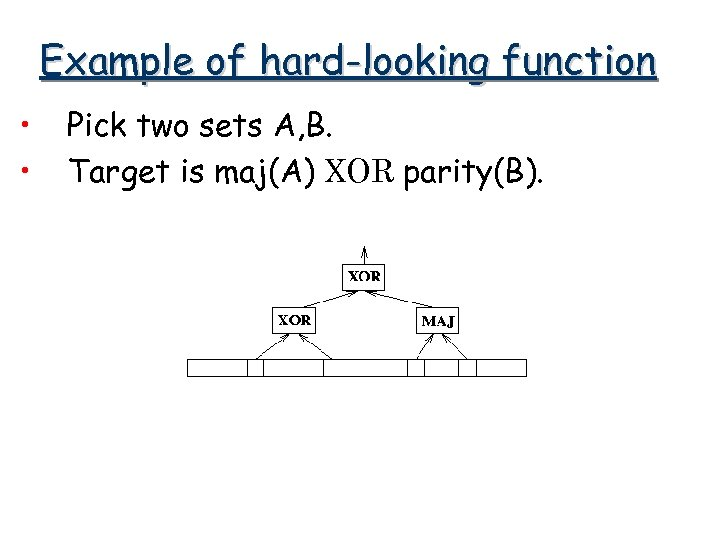Example of hard-looking function • • Pick two sets A, B. Target is maj(A) XOR parity(B).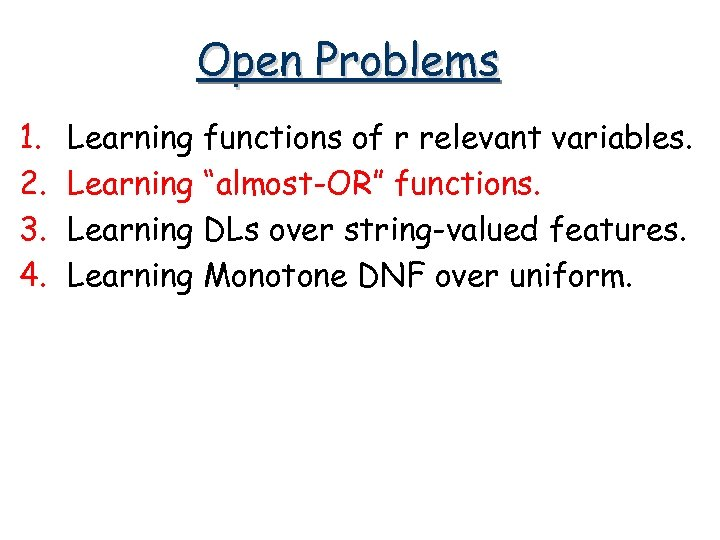Open Problems 1. 2. 3. 4. Learning functions of r relevant variables. Learning “almost-OR” functions. Learning DLs over string-valued features. Learning Monotone DNF over uniform.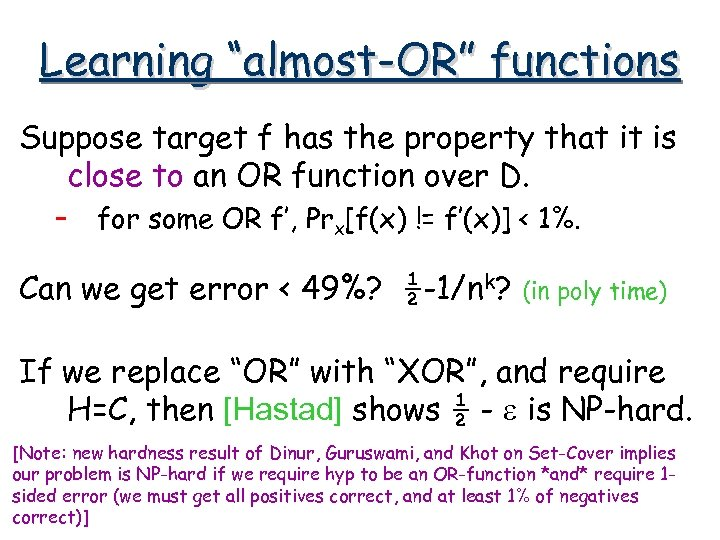Learning “almost-OR” functions Suppose target f has the property that it is close to an OR function over D. – for some OR f’, Prx[f(x) != f’(x)] < 1%. Can we get error < 49%? ½-1/nk? (in poly time) If we replace “OR” with “XOR”, and require H=C, then [Hastad] shows ½ - e is NP-hard. [Note: new hardness result of Dinur, Guruswami, and Khot on Set-Cover implies our problem is NP-hard if we require hyp to be an OR-function *and* require 1 sided error (we must get all positives correct, and at least 1% of negatives correct)]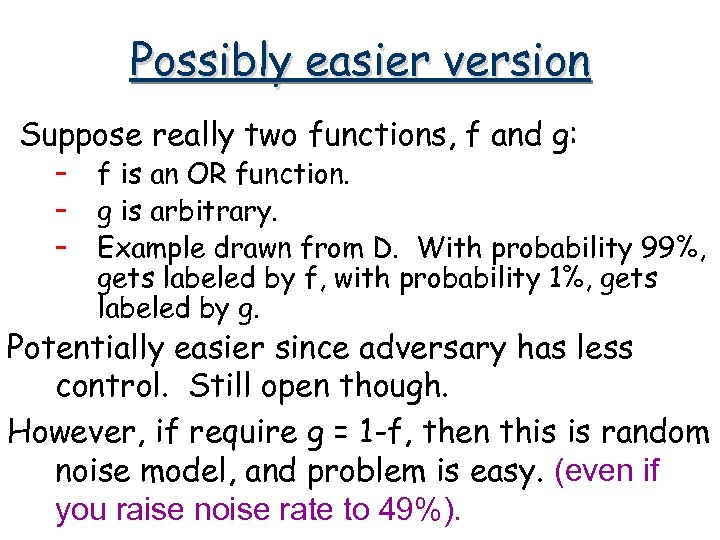Possibly easier version Suppose really two functions, f and g: – – – f is an OR function. g is arbitrary. Example drawn from D. With probability 99%, gets labeled by f, with probability 1%, gets labeled by g. Potentially easier since adversary has less control. Still open though. However, if require g = 1 -f, then this is random noise model, and problem is easy. (even if you raise noise rate to 49%).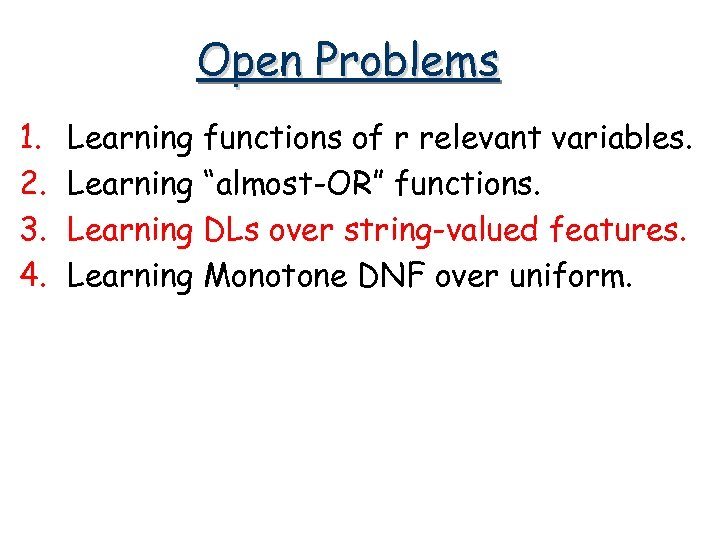Open Problems 1. 2. 3. 4. Learning functions of r relevant variables. Learning “almost-OR” functions. Learning DLs over string-valued features. Learning Monotone DNF over uniform.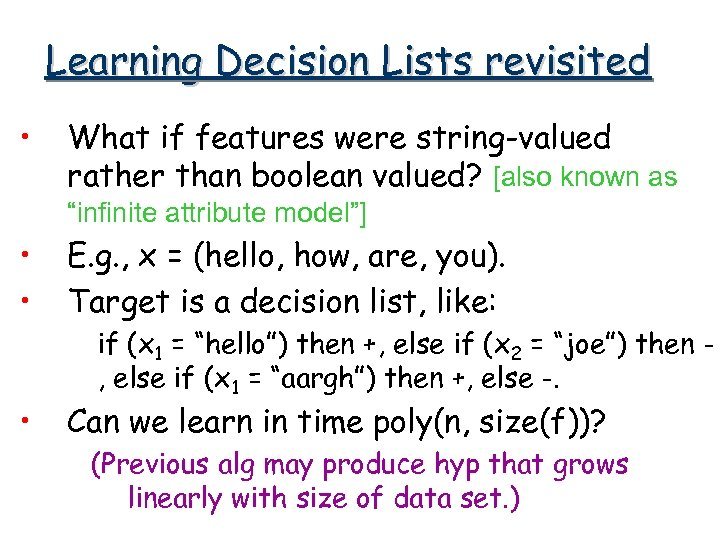Learning Decision Lists revisited • What if features were string-valued rather than boolean valued? [also known as “infinite attribute model”] • • • E. g. , x = (hello, how, are, you). Target is a decision list, like: if (x 1 = “hello”) then +, else if (x 2 = “joe”) then , else if (x 1 = “aargh”) then +, else -. Can we learn in time poly(n, size(f))? (Previous alg may produce hyp that grows linearly with size of data set. )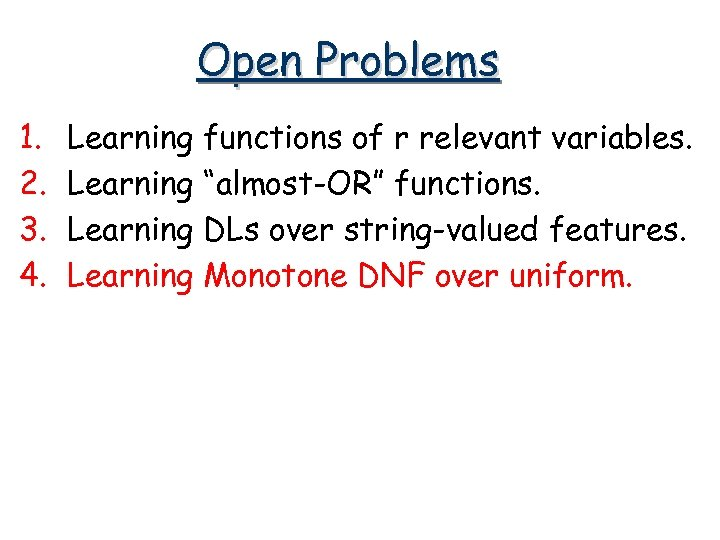Open Problems 1. 2. 3. 4. Learning functions of r relevant variables. Learning “almost-OR” functions. Learning DLs over string-valued features. Learning Monotone DNF over uniform.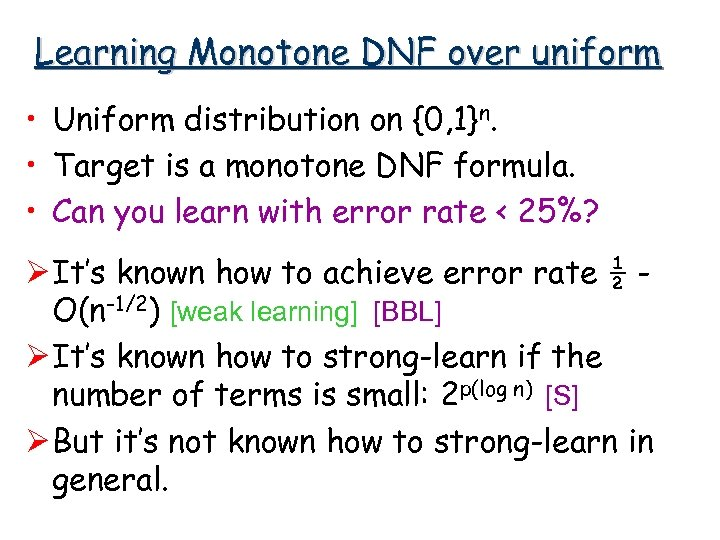Learning Monotone DNF over uniform • Uniform distribution on {0, 1}n. • Target is a monotone DNF formula. • Can you learn with error rate < 25%? Ø It’s known how to achieve error rate ½ O(n-1/2) [weak learning] [BBL] Ø It’s known how to strong-learn if the number of terms is small: 2 p(log n) [S] Ø But it’s not known how to strong-learn in general.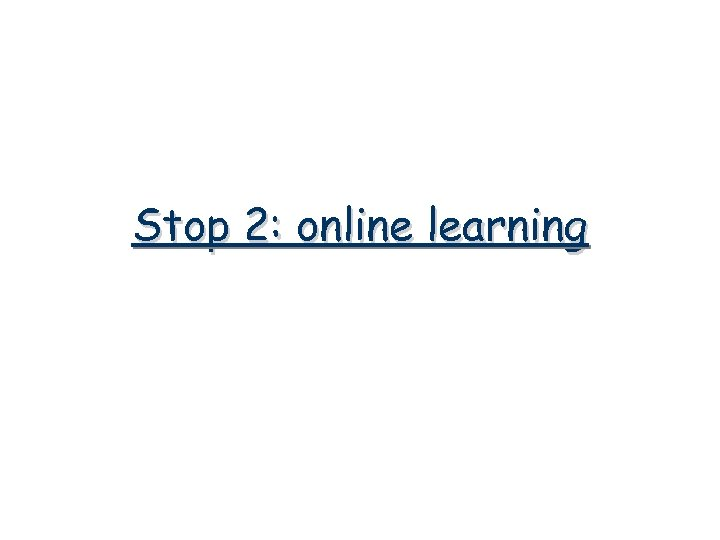Stop 2: online learning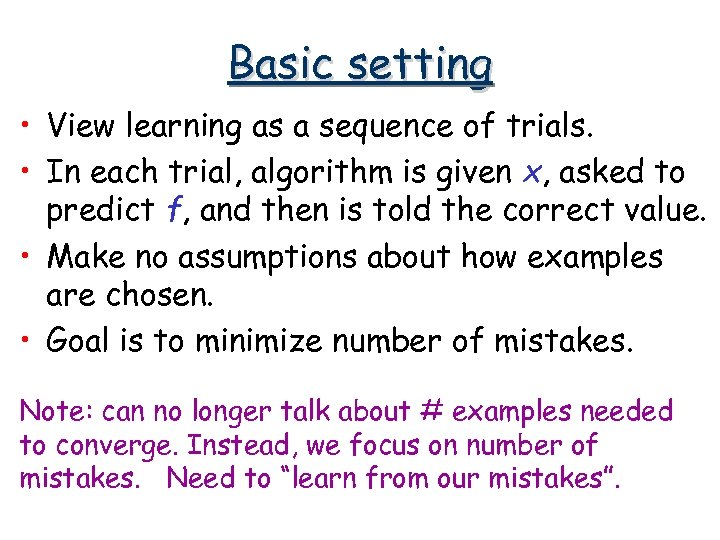Basic setting • View learning as a sequence of trials. • In each trial, algorithm is given x, asked to predict f, and then is told the correct value. • Make no assumptions about how examples are chosen. • Goal is to minimize number of mistakes. Note: can no longer talk about # examples needed to converge. Instead, we focus on number of mistakes. Need to “learn from our mistakes”.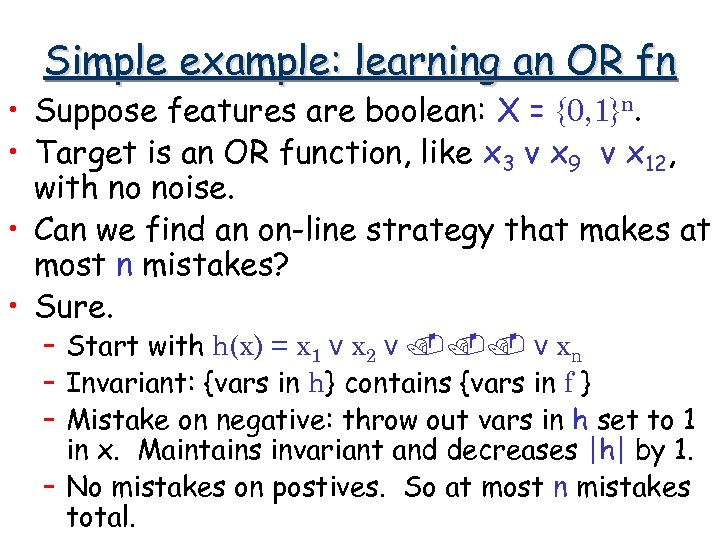Simple example: learning an OR fn • Suppose features are boolean: X = {0, 1}n. • Target is an OR function, like x 3 v x 9 v x 12, with no noise. • Can we find an on-line strategy that makes at most n mistakes? • Sure. – Start with h(x) = x 1 v x 2 v. . . v xn – Invariant: {vars in h} contains {vars in f } – Mistake on negative: throw out vars in h set to 1 in x. Maintains invariant and decreases |h| by 1. – No mistakes on postives. So at most n mistakes total.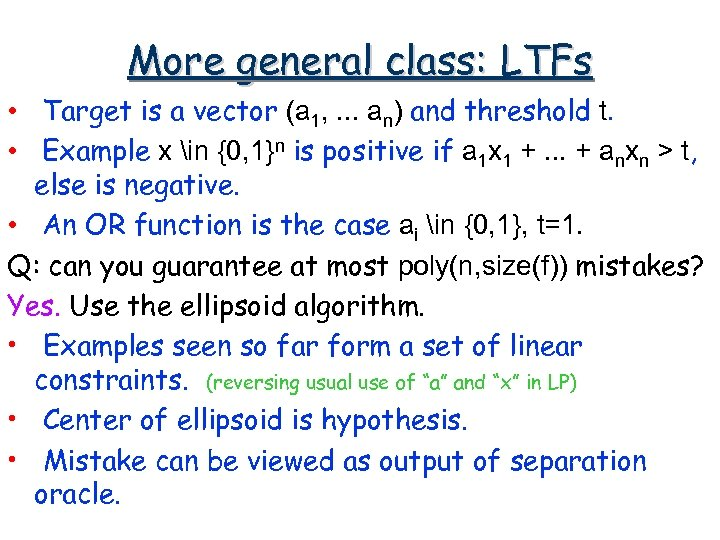More general class: LTFs • Target is a vector (a 1, . . . an) and threshold t. • Example x in {0, 1}n is positive if a 1 x 1 +. . . + anxn > t, else is negative. • An OR function is the case ai in {0, 1}, t=1. Q: can you guarantee at most poly(n, size(f)) mistakes? Yes. Use the ellipsoid algorithm. • Examples seen so far form a set of linear constraints. (reversing usual use of “a” and “x” in LP) • Center of ellipsoid is hypothesis. • Mistake can be viewed as output of separation oracle.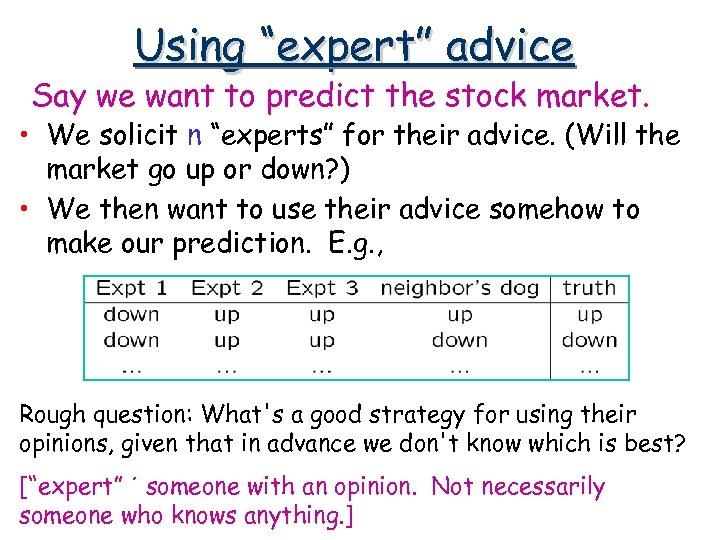Using “expert” advice Say we want to predict the stock market. • We solicit n “experts” for their advice. (Will the market go up or down? ) • We then want to use their advice somehow to make our prediction. E. g. , Rough question: What's a good strategy for using their opinions, given that in advance we don't know which is best? [“expert” ´ someone with an opinion. Not necessarily someone who knows anything. ]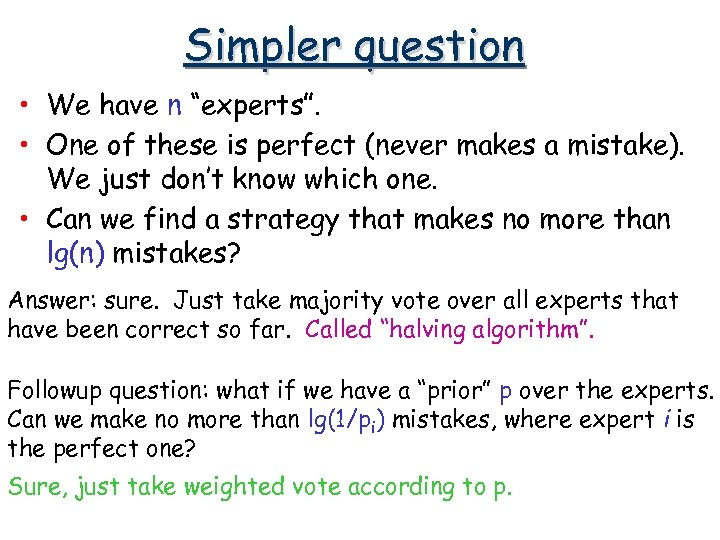Simpler question • We have n “experts”. • One of these is perfect (never makes a mistake). We just don’t know which one. • Can we find a strategy that makes no more than lg(n) mistakes? Answer: sure. Just take majority vote over all experts that have been correct so far. Called “halving algorithm”. Followup question: what if we have a “prior” p over the experts. Can we make no more than lg(1/pi) mistakes, where expert i is the perfect one? Sure, just take weighted vote according to p.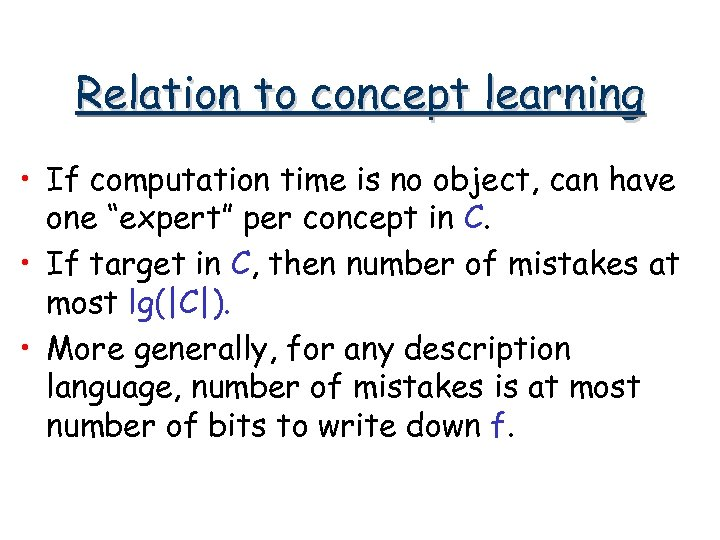Relation to concept learning • If computation time is no object, can have one “expert” per concept in C. • If target in C, then number of mistakes at most lg(|C|). • More generally, for any description language, number of mistakes is at most number of bits to write down f.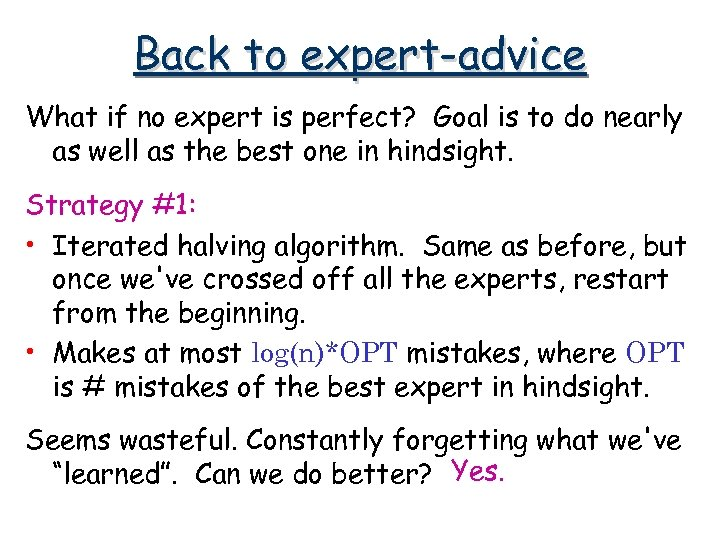Back to expert-advice What if no expert is perfect? Goal is to do nearly as well as the best one in hindsight. Strategy #1: • Iterated halving algorithm. Same as before, but once we've crossed off all the experts, restart from the beginning. • Makes at most log(n)*OPT mistakes, where OPT is # mistakes of the best expert in hindsight. Seems wasteful. Constantly forgetting what we've “learned”. Can we do better? Yes.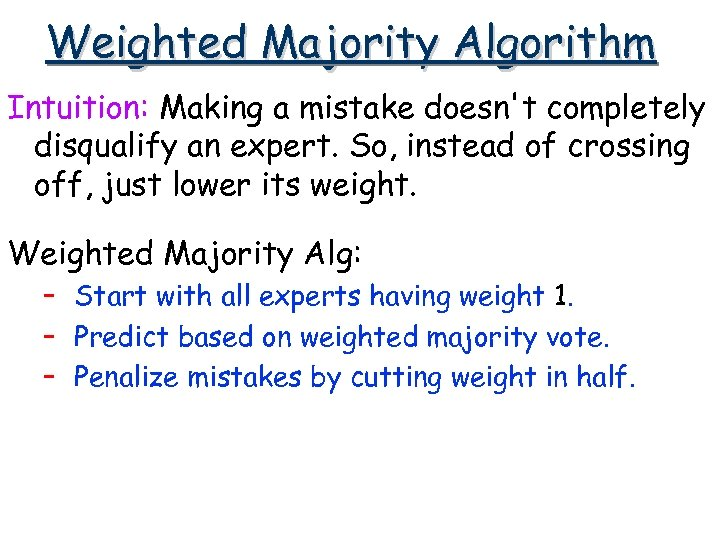Weighted Majority Algorithm Intuition: Making a mistake doesn't completely disqualify an expert. So, instead of crossing off, just lower its weight. Weighted Majority Alg: – Start with all experts having weight 1. – Predict based on weighted majority vote. – Penalize mistakes by cutting weight in half.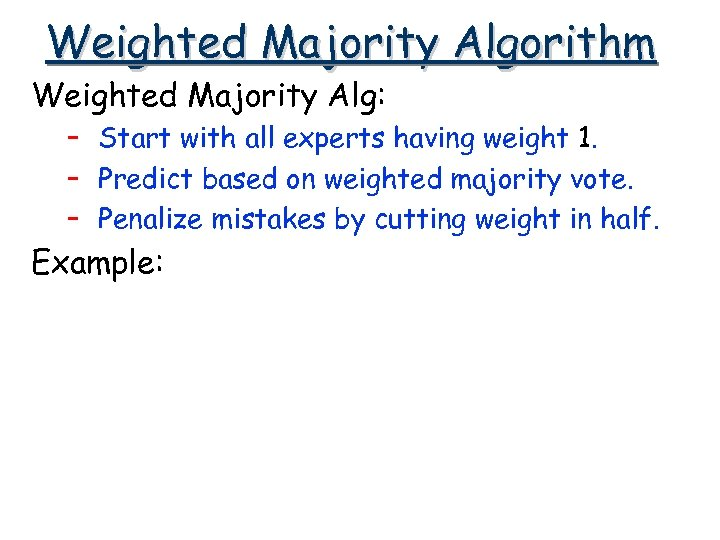Weighted Majority Algorithm Weighted Majority Alg: – Start with all experts having weight 1. – Predict based on weighted majority vote. – Penalize mistakes by cutting weight in half. Example: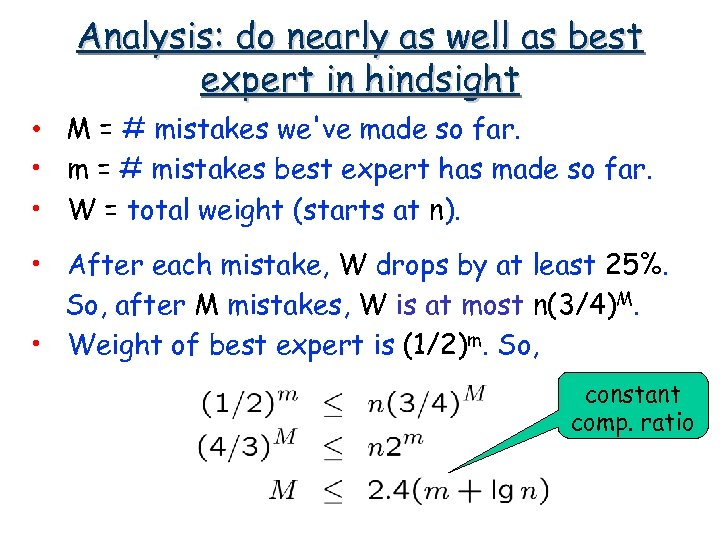Analysis: do nearly as well as best expert in hindsight • M = # mistakes we've made so far. • m = # mistakes best expert has made so far. • W = total weight (starts at n). • After each mistake, W drops by at least 25%. So, after M mistakes, W is at most n(3/4)M. • Weight of best expert is (1/2)m. So, constant comp. ratio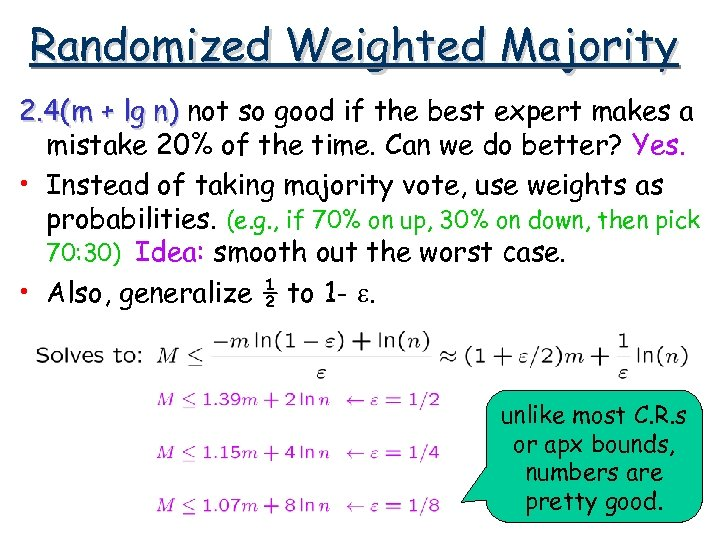Randomized Weighted Majority 2. 4(m + lg n) not so good if the best expert makes a mistake 20% of the time. Can we do better? Yes. • Instead of taking majority vote, use weights as probabilities. (e. g. , if 70% on up, 30% on down, then pick 70: 30) Idea: smooth out the worst case. • Also, generalize ½ to 1 - e. unlike most C. R. s or apx bounds, numbers are pretty good.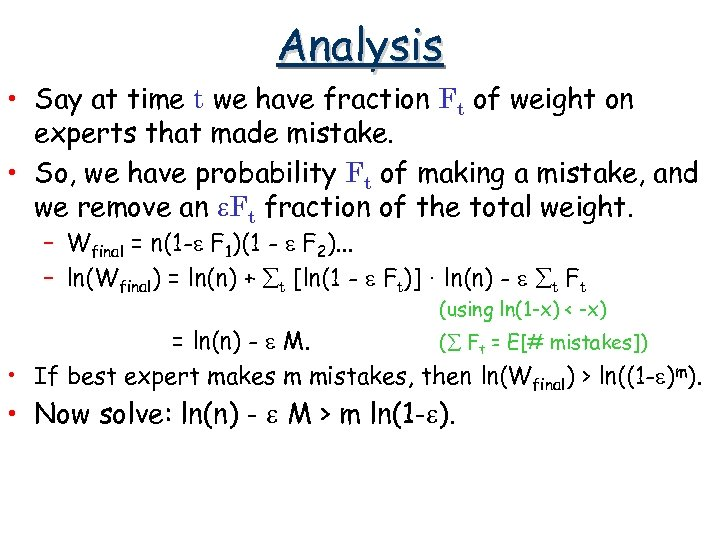Analysis • Say at time t we have fraction Ft of weight on experts that made mistake. • So, we have probability Ft of making a mistake, and we remove an e. Ft fraction of the total weight. – Wfinal = n(1 -e F 1)(1 - e F 2). . . – ln(Wfinal) = ln(n) + åt [ln(1 - e Ft)] · ln(n) - e åt Ft (using ln(1 -x) < -x) = ln(n) - e M. (å Ft = E[# mistakes]) • If best expert makes m mistakes, then ln(Wfinal) > ln((1 -e)m). • Now solve: ln(n) - e M > m ln(1 -e).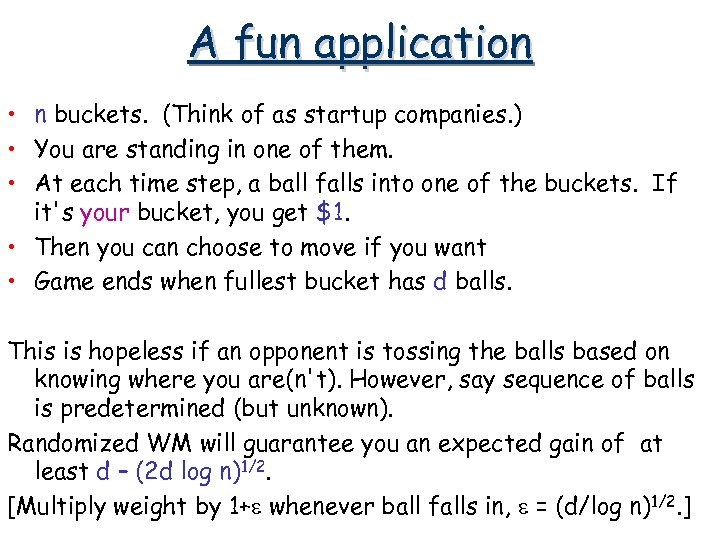A fun application • n buckets. (Think of as startup companies. ) • You are standing in one of them. • At each time step, a ball falls into one of the buckets. If it's your bucket, you get \$1. • Then you can choose to move if you want • Game ends when fullest bucket has d balls. This is hopeless if an opponent is tossing the balls based on knowing where you are(n't). However, say sequence of balls is predetermined (but unknown). Randomized WM will guarantee you an expected gain of at least d – (2 d log n)1/2. [Multiply weight by 1+e whenever ball falls in, e = (d/log n)1/2. ]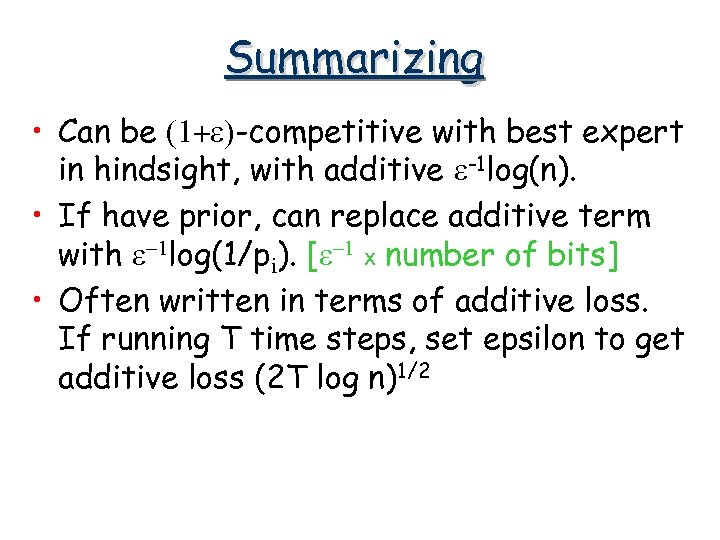Summarizing • Can be (1+e)-competitive with best expert in hindsight, with additive e-1 log(n). • If have prior, can replace additive term with e-1 log(1/pi). [e-1 x number of bits] • Often written in terms of additive loss. If running T time steps, set epsilon to get additive loss (2 T log n)1/2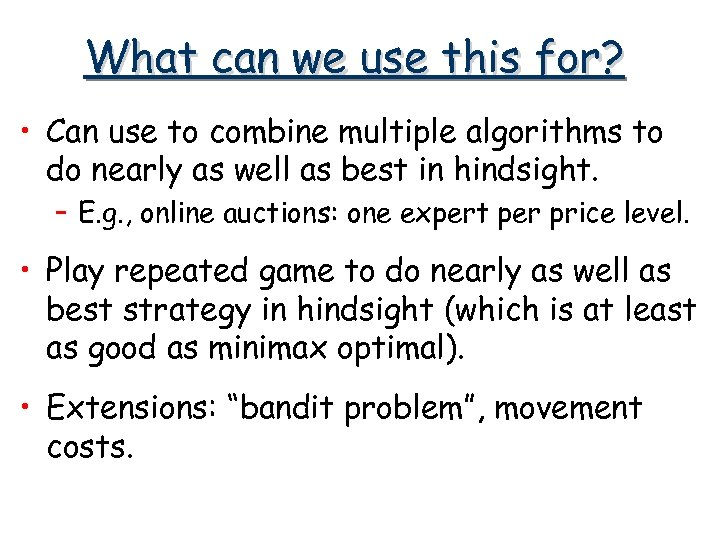What can we use this for? • Can use to combine multiple algorithms to do nearly as well as best in hindsight. – E. g. , online auctions: one expert per price level. • Play repeated game to do nearly as well as best strategy in hindsight (which is at least as good as minimax optimal). • Extensions: “bandit problem”, movement costs.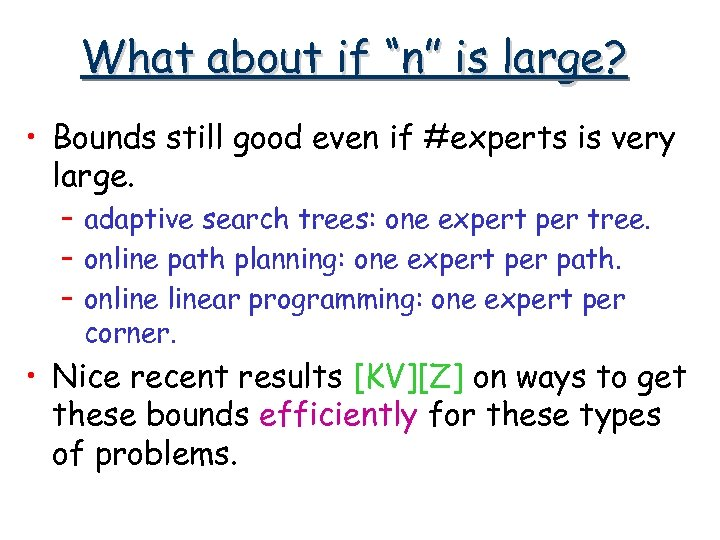What about if “n” is large? • Bounds still good even if #experts is very large. – adaptive search trees: one expert per tree. – online path planning: one expert per path. – onlinear programming: one expert per corner. • Nice recent results [KV][Z] on ways to get these bounds efficiently for these types of problems.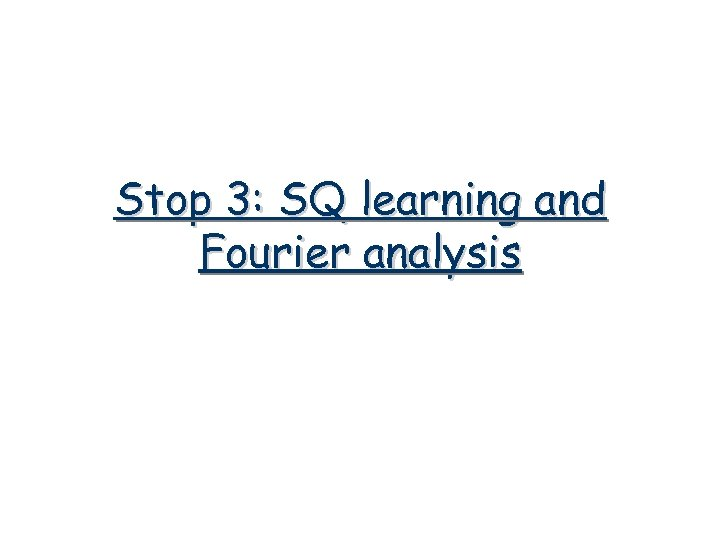Stop 3: SQ learning and Fourier analysis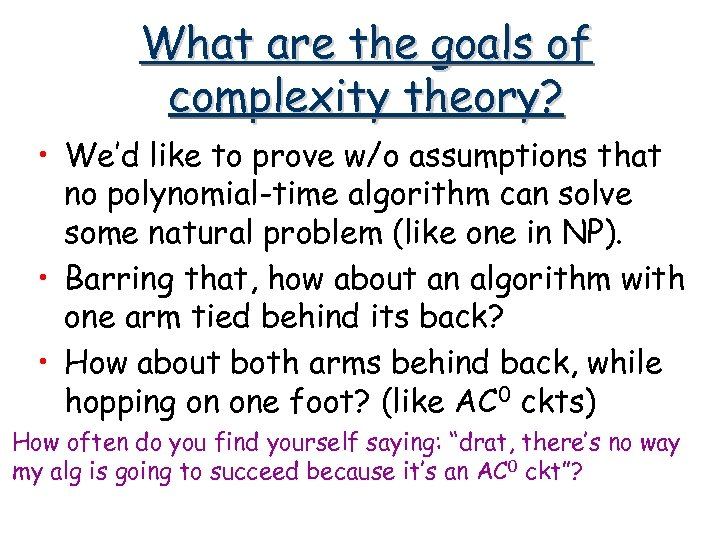What are the goals of complexity theory? • We’d like to prove w/o assumptions that no polynomial-time algorithm can solve some natural problem (like one in NP). • Barring that, how about an algorithm with one arm tied behind its back? • How about both arms behind back, while hopping on one foot? (like AC 0 ckts) How often do you find yourself saying: “drat, there’s no way my alg is going to succeed because it’s an AC 0 ckt”?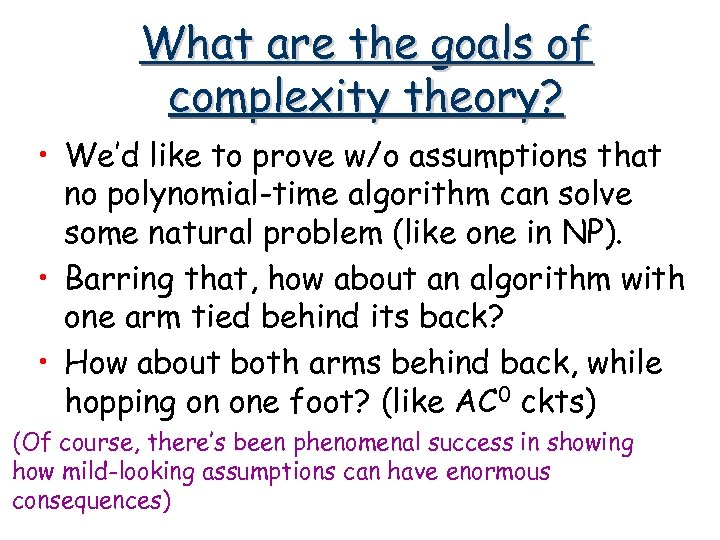What are the goals of complexity theory? • We’d like to prove w/o assumptions that no polynomial-time algorithm can solve some natural problem (like one in NP). • Barring that, how about an algorithm with one arm tied behind its back? • How about both arms behind back, while hopping on one foot? (like AC 0 ckts) (Of course, there’s been phenomenal success in showing how mild-looking assumptions can have enormous consequences)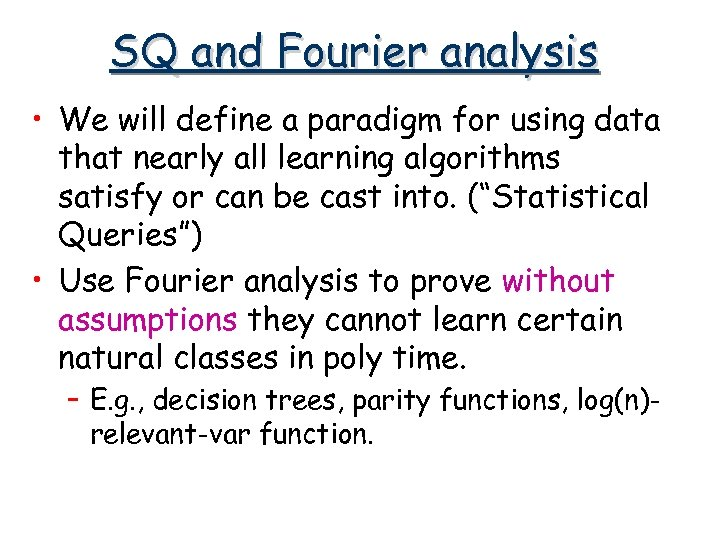SQ and Fourier analysis • We will define a paradigm for using data that nearly all learning algorithms satisfy or can be cast into. (“Statistical Queries”) • Use Fourier analysis to prove without assumptions they cannot learn certain natural classes in poly time. – E. g. , decision trees, parity functions, log(n)relevant-var function.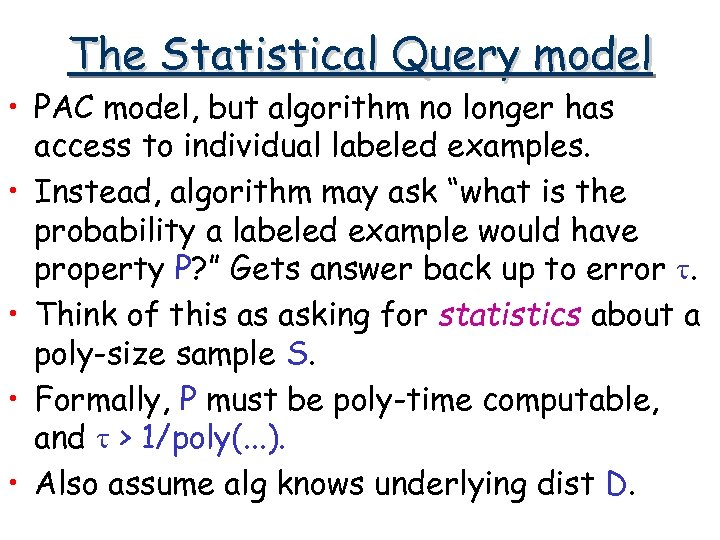The Statistical Query model • PAC model, but algorithm no longer has access to individual labeled examples. • Instead, algorithm may ask “what is the probability a labeled example would have property P? ” Gets answer back up to error t. • Think of this as asking for statistics about a poly-size sample S. • Formally, P must be poly-time computable, and t > 1/poly(. . . ). • Also assume alg knows underlying dist D.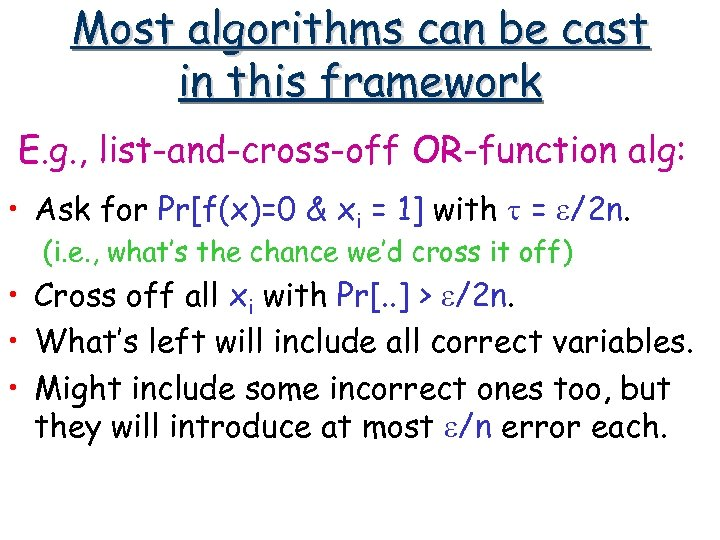Most algorithms can be cast in this framework E. g. , list-and-cross-off OR-function alg: • Ask for Pr[f(x)=0 & xi = 1] with t = e/2 n. (i. e. , what’s the chance we’d cross it off) • Cross off all xi with Pr[. . ] > e/2 n. • What’s left will include all correct variables. • Might include some incorrect ones too, but they will introduce at most e/n error each.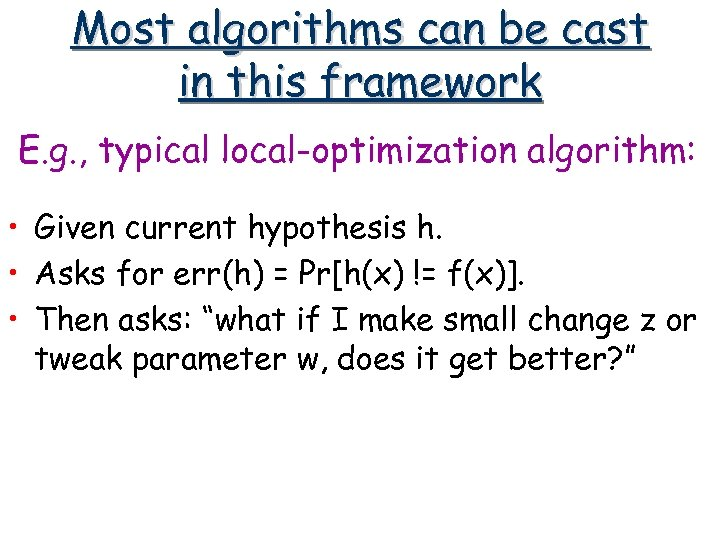Most algorithms can be cast in this framework E. g. , typical local-optimization algorithm: • Given current hypothesis h. • Asks for err(h) = Pr[h(x) != f(x)]. • Then asks: “what if I make small change z or tweak parameter w, does it get better? ”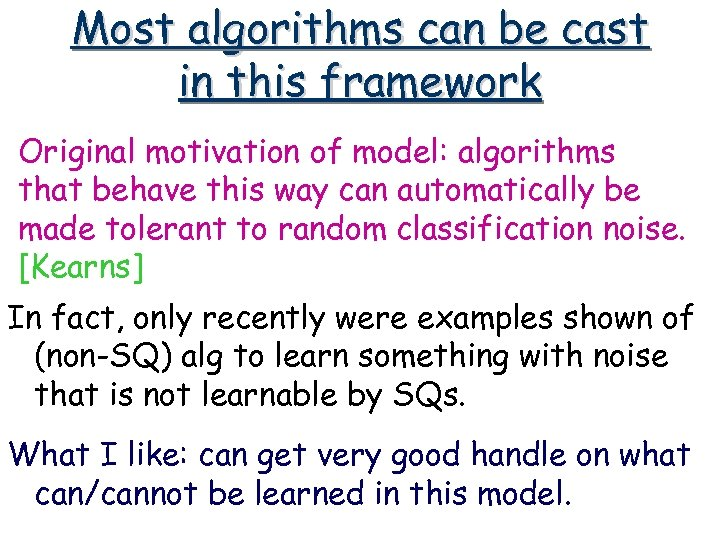Most algorithms can be cast in this framework Original motivation of model: algorithms that behave this way can automatically be made tolerant to random classification noise. [Kearns] In fact, only recently were examples shown of (non-SQ) alg to learn something with noise that is not learnable by SQs. What I like: can get very good handle on what can/cannot be learned in this model.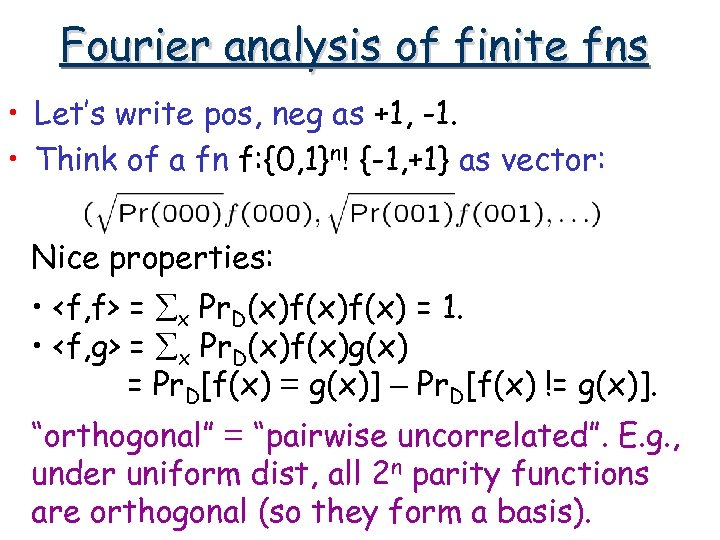Fourier analysis of finite fns • Let’s write pos, neg as +1, -1. • Think of a fn f: {0, 1}n! {-1, +1} as vector: Nice properties: • = åx Pr. D(x)f(x) = 1. • = åx Pr. D(x)f(x)g(x) = Pr. D[f(x) = g(x)] – Pr. D[f(x) != g(x)]. “orthogonal” = “pairwise uncorrelated”. E. g. , under uniform dist, all 2 n parity functions are orthogonal (so they form a basis).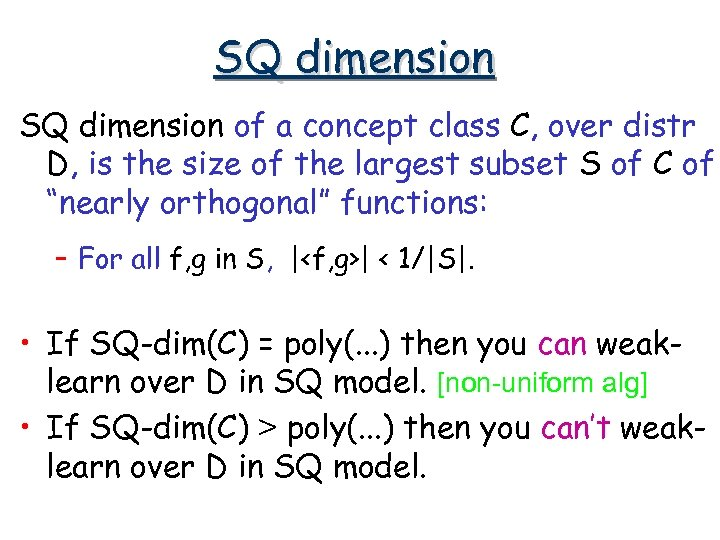SQ dimension of a concept class C, over distr D, is the size of the largest subset S of C of “nearly orthogonal” functions: – For all f, g in S, || < 1/|S|. • If SQ-dim(C) = poly(. . . ) then you can weaklearn over D in SQ model. [non-uniform alg] • If SQ-dim(C) > poly(. . . ) then you can’t weaklearn over D in SQ model.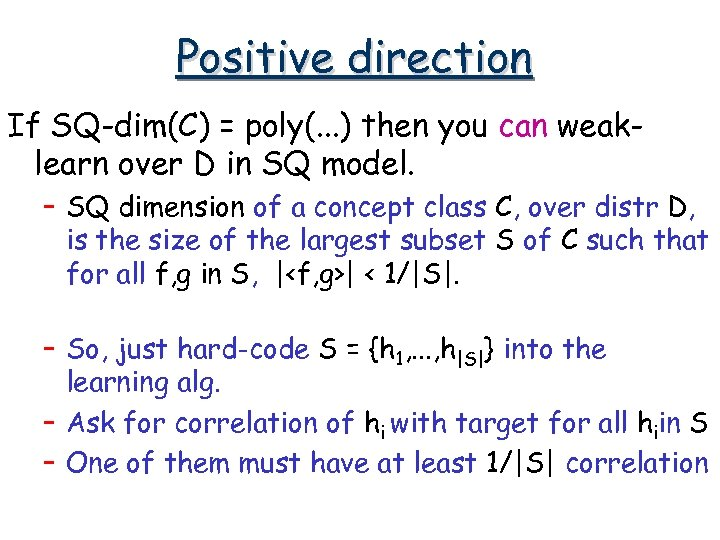Positive direction If SQ-dim(C) = poly(. . . ) then you can weaklearn over D in SQ model. – SQ dimension of a concept class C, over distr D, is the size of the largest subset S of C such that for all f, g in S, || < 1/|S|. – So, just hard-code S = {h 1, . . . , h|S|} into the learning alg. – Ask for correlation of hi with target for all hiin S – One of them must have at least 1/|S| correlation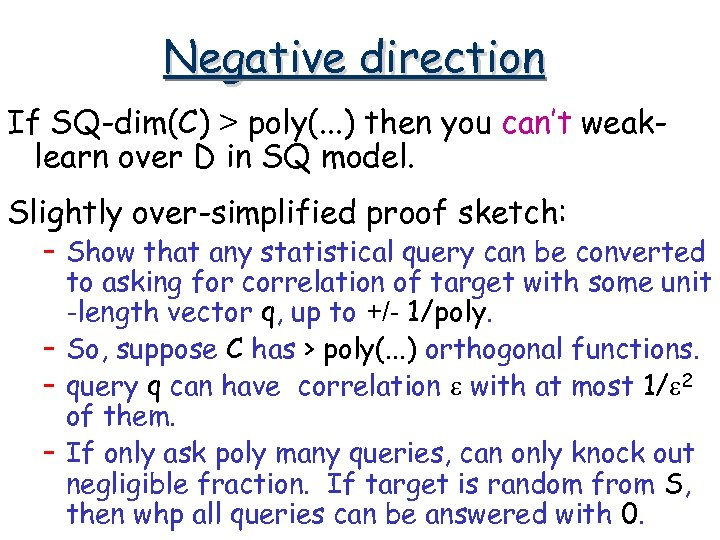Negative direction If SQ-dim(C) > poly(. . . ) then you can’t weaklearn over D in SQ model. Slightly over-simplified proof sketch: – Show that any statistical query can be converted to asking for correlation of target with some unit -length vector q, up to +/- 1/poly. – So, suppose C has > poly(. . . ) orthogonal functions. – query q can have correlation e with at most 1/e 2 of them. – If only ask poly many queries, can only knock out negligible fraction. If target is random from S, then whp all queries can be answered with 0.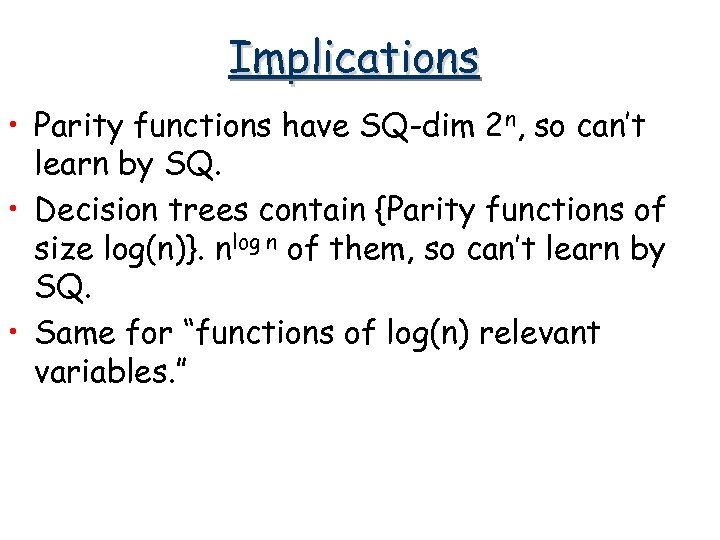Implications • Parity functions have SQ-dim 2 n, so can’t learn by SQ. • Decision trees contain {Parity functions of size log(n)}. nlog n of them, so can’t learn by SQ. • Same for “functions of log(n) relevant variables. ”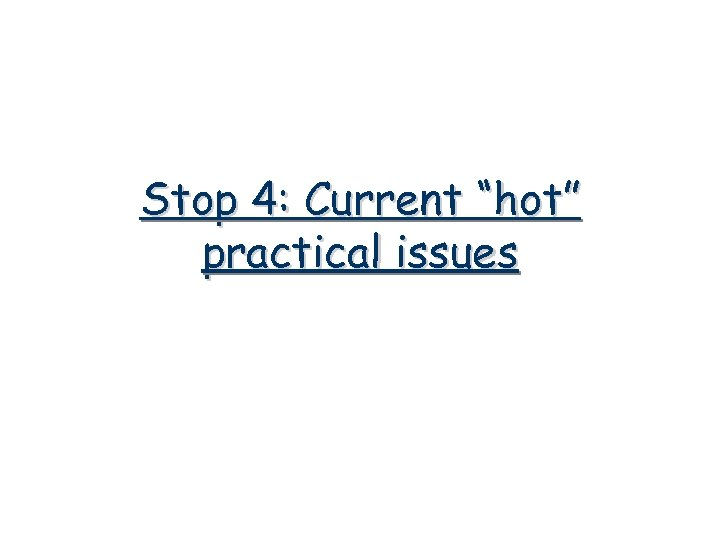Stop 4: Current “hot” practical issues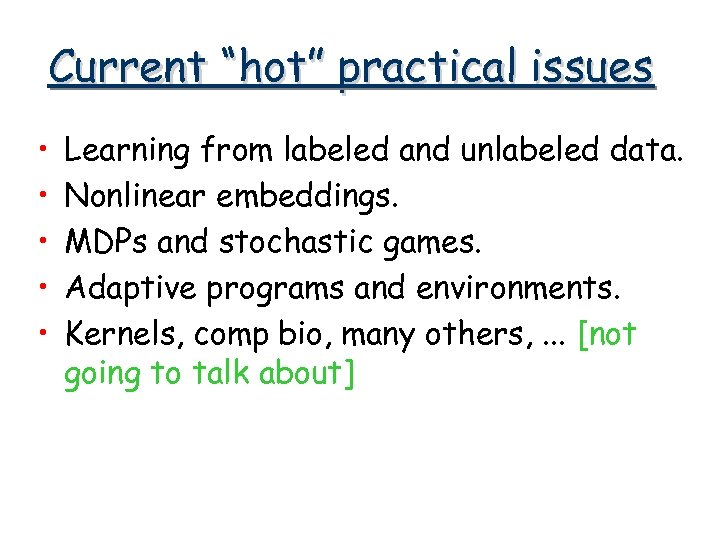Current “hot” practical issues • • • Learning from labeled and unlabeled data. Nonlinear embeddings. MDPs and stochastic games. Adaptive programs and environments. Kernels, comp bio, many others, . . . [not going to talk about]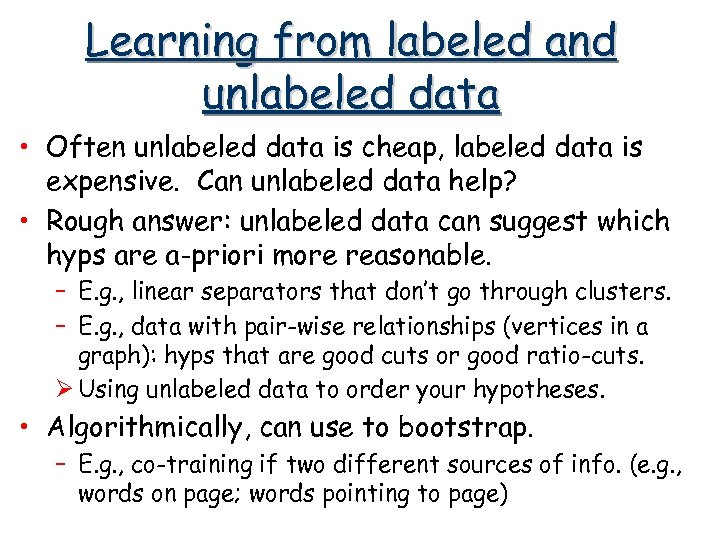Learning from labeled and unlabeled data • Often unlabeled data is cheap, labeled data is expensive. Can unlabeled data help? • Rough answer: unlabeled data can suggest which hyps are a-priori more reasonable. – E. g. , linear separators that don’t go through clusters. – E. g. , data with pair-wise relationships (vertices in a graph): hyps that are good cuts or good ratio-cuts. Ø Using unlabeled data to order your hypotheses. • Algorithmically, can use to bootstrap. – E. g. , co-training if two different sources of info. (e. g. , words on page; words pointing to page)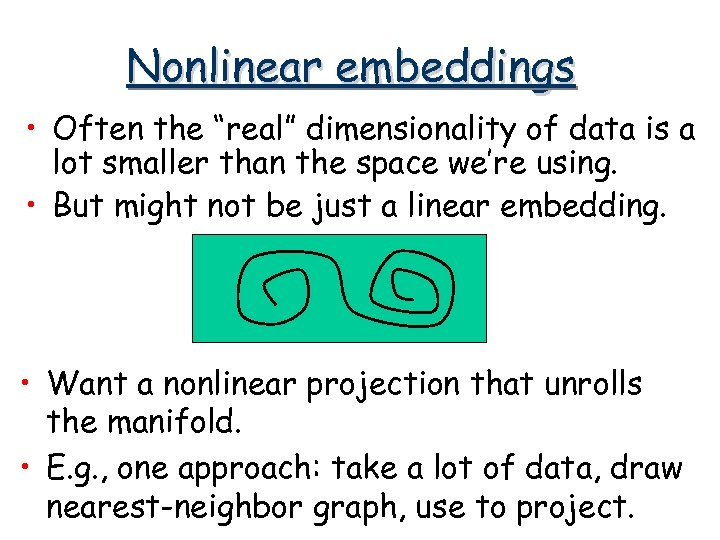Nonlinear embeddings • Often the “real” dimensionality of data is a lot smaller than the space we’re using. • But might not be just a linear embedding. • Want a nonlinear projection that unrolls the manifold. • E. g. , one approach: take a lot of data, draw nearest-neighbor graph, use to project.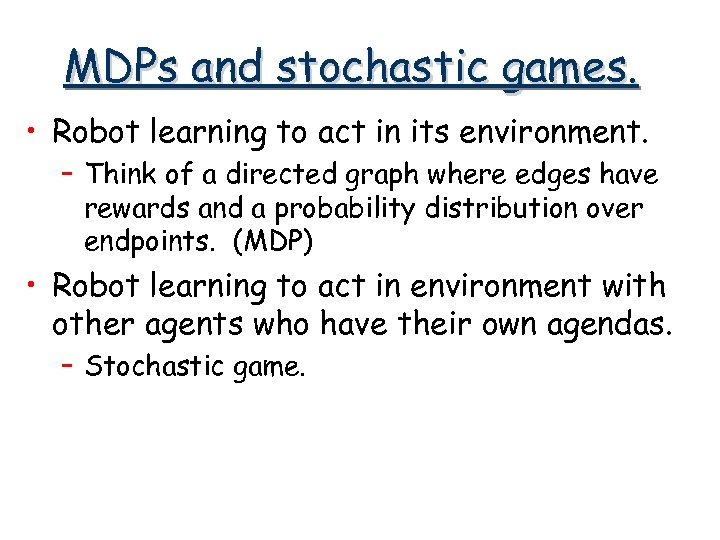MDPs and stochastic games. • Robot learning to act in its environment. – Think of a directed graph where edges have rewards and a probability distribution over endpoints. (MDP) • Robot learning to act in environment with other agents who have their own agendas. – Stochastic game.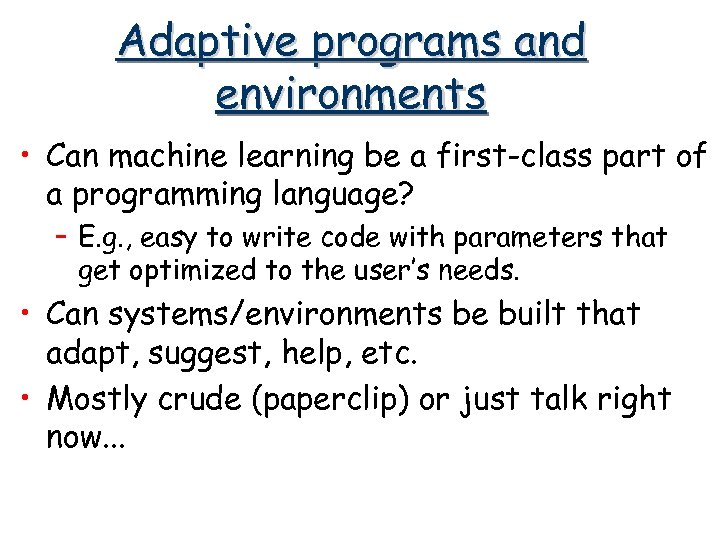Adaptive programs and environments • Can machine learning be a first-class part of a programming language? – E. g. , easy to write code with parameters that get optimized to the user’s needs. • Can systems/environments be built that adapt, suggest, help, etc. • Mostly crude (paperclip) or just talk right now. . .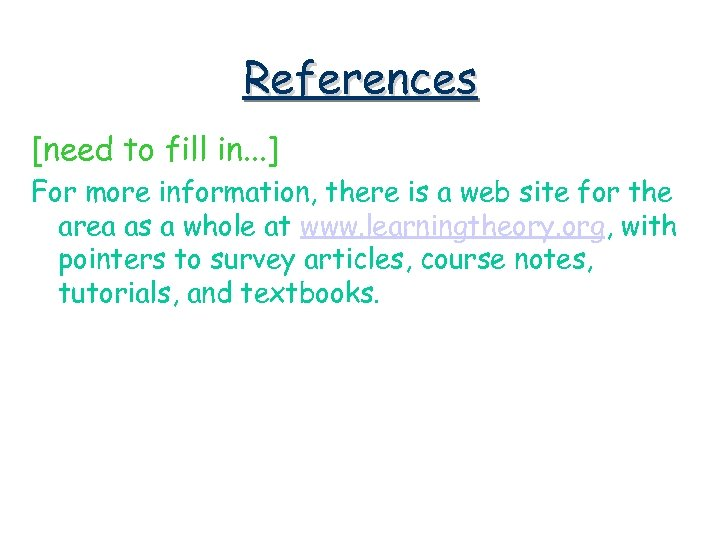References [need to fill in. . . ] For more information, there is a web site for the area as a whole at www. learningtheory. org, with pointers to survey articles, course notes, tutorials, and textbooks.